## How to Get Row Number From a Vlookup in Excel

This post will guide you how to get row number from a Vlookup in Excel. How do I return a row number from a Vlookup with a formula in Excel.

Assuming that you have a list of data in range A1:B5, you can use the VLOOKUP function to lookup a value in the first column and then return the corresponding cell value in the same row. But now I do not want to get the cell value, and I want to get the row number from a VLookup in Excel. How to accomplish it.

## 1. Get Row Number From a Vlookup

If you want to return a row number from a Vlookup, you can use a formula based on the MATCH function. For example, you want to lookup a string value “excel” in range A1:A5 and return the row number where the searching value is found. Like this:

``=MATCH("excel",A2:A5,0)``

You need to type this formula into a desired cell and press Enter key to apply this formula. You would see that the row number returned.

Note: the above formula only returns a relative row number, and if you want to get a absolute row number, you can use another formula based on the ROW function and the MATCH function, like this:

``=MATCH("excel",A1:A5,0) + ROW(A1:A5)-1``

Type this formula into a blank cell and press Enter key to apply it.

## 2. Video: Get Row Number from a VLOOKUP in Excel

This video will show you how to use the MATCH function to get a relative row number and how to combine it with the ROW function to get an absolute row number from a VLOOKUP in Excel.

## 3. Related Functions

• Excel VLOOKUP function
The Excel VLOOKUP function lookup a value in the first column of the table and return the value in the same row based on index_num position.The syntax of the VLOOKUP function is as below:= VLOOKUP (lookup_value, table_array, column_index_num,[range_lookup])….
• Excel ROW function
The Excel ROW function returns the row number of a cell reference.The ROW function is a build-in function in Microsoft Excel and it is categorized as a Lookup and Reference Function.The syntax of the ROW function is as below:= ROW ([reference])….
• Excel MATCH function
The Excel MATCH function search a value in an array and returns the position of that item.The syntax of the MATCH function is as below:= MATCH  (lookup_value, lookup_array, [match_type])….

## How to Count Only Unique Values Excluding Duplicates in Excel

We enter a list of numbers or products and there are some duplicates in the list, if we want to just do count for the unique values and exclude the duplicates, how can we do? Now you can follow the below steps to solve this question by formula quickly.

Prepare a list of products and there are some duplicates among the list. See example below:

And we want to count the total product type in one year, how can we do count?

If we just use sum formula to do count, then duplicates will be included, so we need another formula to do count excludes the duplicates. See steps below.

## 1. Count Unique Values Excluding Duplicates by Formula in Excel

Step 1: In E2 which is saved the total product type number, enter the formula:

``=SUMPRODUCT(1/COUNTIF(B1:B11,B1:B11))``

Where B1:B11 is the range you want to count the unique values.

Step 2: Click Enter and get the result in E2. We can check the result is 6, and the duplicates are not included.

In above sample, we do count for unique values and get the result 6, because we have six products A\B\C\D\E\F, and if we want to only count the unique values exclude all duplicates like product A\B\C (they appeared more than one season) how can we do count? See below steps.

## 2. Count Unique Values Excluding All Duplicates by Formula in Excel

Step 1: In E2 which is saved the total product type number, enter the formula:

``=SUM(IF(FREQUENCY(MATCH(B1:B11,B1:B11,0),ROW(B1:B11)-ROW(B1)+1)=1,1))``

Where B1:B11 is the range you want to count the unique values.

Step2: Click Enter and get the result in E2. We can check the result is 3 (Product D\E\F are unique values and only appeared in one season).

## 3. Video: Count Only Unique Values Excluding Duplicates

If you want to learn how to count only unique values excluding duplicates in Excel, this video will show you a simple and effective formula that you can use in any situation.

## 4. Related Functions

• Excel SUMPRODUCT function
The Excel SUMPRODUCT function multiplies corresponding components in the given one or more arrays or ranges, and returns the sum of those products.The syntax of the SUMPRODUCT function is as below:= SUMPRODUCT (array1,[array2],…)…
• Excel COUNTIF function
The Excel COUNTIF function will count the number of cells in a range that meet a given criteria. This function can be used to count the different kinds of cells with number, date, text values, blank, non-blanks, or containing specific characters.etc.= COUNTIF (range, criteria)…
• Excel ROW function
The Excel ROW function returns the row number of a cell reference.The ROW function is a build-in function in Microsoft Excel and it is categorized as a Lookup and Reference Function.The syntax of the ROW function is as below:= ROW ([reference])….
• Excel MATCH function
The Excel MATCH function search a value in an array and returns the position of that item.The syntax of the MATCH function is as below:= MATCH  (lookup_value, lookup_array, [match_type])…
• Excel IF function
The Excel IF function perform a logical test to return one value if the condition is TRUE and return another value if the condition is FALSE. The IF function is a build-in function in Microsoft Excel and it is categorized as a Logical Function.The syntax of the IF function is as below:= IF (condition, [true_value], [false_value])….
• Excel SUM function
The Excel SUM function will adds all numbers in a range of cells and returns the sum of these values. You can add individual values, cell references or ranges in excel.The syntax of the SUM function is as below:= SUM(number1,[number2],…)…

## Excel Array Construction

This article will talk about how to create one-dimensional array or two-dimensional array by using some functions in Excel. When using array formulas in Excel, we often use functions to construct arrays.

## 1. Generate Array with ROW or COLUMN Functions

Array formulas often need to use “natural number” as parameters of the function, such as the second parameter of the LARGE function, OFFSET function in addition to the first parameter. Through the manual way to enter a constant array will be more trouble, and easy to make mistakes. Then we can use the ROW or COLUMN function in EXCEL to generate a sequence, this method is very convenient and fast.

The following formula produces a vertical array of natural numbers from `1` to `10`.

``{=ROW (1:10)}``

The following formula produces a horizontal array of natural numbers from `1 `to `10`.

``{=COLUMN(A:J)}``

## 2. Generating two-dimensional Array from one-dimensional Array

Below we will show you how to construct a new two-dimensional array with two columns of data.

### a. One-dimensional range rearrangement to generate two-dimensional array

If there is a list of students and the name column of that list contains 10 students’ names, we need to randomly place the students’ names in the name column into the cell range of `5 rows and 2 columns` (a new two-dimensional array).

We can use the following steps to randomize the names of students in `column B` to a `5 row 2 column` range, such as the cell range `E1:F5`.

STEP1# Select the cell range `E1:F5`

STEP2# Enter the following array formula in the formula bar

``=INDEX(B2:B11,RIGHT(SMALL(RANDBETWEEN(A2:A11^0,999)/1%+A2:A11,ROW(1:5)*2-{1,0}),2))``

STEP3# Press CTRL + SHIFT +ENTER shortcut keys to convert the above formula into an array formula.

STEP4# You will see that the students’ names have been randomly placed in a two-dimensional range of array.

Let’s see how this array formula works.

=RANDBETWEEN(A2:A11^0,999)

The RANDBETWEEN function is used to generate an array of 10 values, where the elements are random integers between` 1` and `1000`. Since the elements are randomly generated, the size of the array elements is randomly ordered. The array formula generates an array of random integers as follows.

``={484;203;468;525;702;220;13;163;386;54}``

=RANDBETWEEN(A2:A11^0,999)/1%+A2:A11

The random integer array generated above is multiplied by `100 `and then added to the ordinal array of `1` to `10`. This ensures that the last two digits of the array elements are ordinal numbers `1 `to `10`.

={484;203;468;525;702;220;13;163;386;54}/1%+A2:A11

The above array formula returns the following array:

``{48401;20302;46803;52504;70205;22006;1307;16308;38609;5410}``

=ROW(1:5)*2-{1,0}

The ROW function generates a vertical array` {1;2;3;4;5)`, and then subtracts a constant array {1,0} to produce a two-dimensional array of 5 rows and 2 columns.

=SMALL()

The result is taken as the second argument to the SMALL function, which sorts the array after multiplication and addition processing. Since the original size of the array is random, after sorting, the ordinal number corresponding to the last two digits of each element is randomly ordered.

=RIGHT()

The RIGHT function is used to extract the last two digits of each element, and the INDEX function is used to return the student’s name in the corresponding position in `column B`. In this way, the names in `column B `can be randomly populated into a two-dimensional array of `5 rows and 2 columns`.

### b. Combining two columns of data to create a two-dimensional array

We can use the VLOOKUP function to query from the right to the left, and we can use the array operation and IF function to swap two columns of data to generate a new two-dimensional array.

Here is an example of how the VLOOKUP function can be used to reverse the query by constructing a new array.

If you have a table of employee information, and you need to find the employee’s number by the employee’s name, you can use the VLOOKUP function in combination with the IF function to construct a two-dimensional array to find the corresponding employee number.

The employee information table is as follows:

To find the employee number by name, the steps are as follows.

STEP1# Select the cell `B3`

STEP2# Enter the following formula in the formula bar and press Enter

``=VLOOKUP(B2,IF({1,0},E2:E11,D2:D11),2,)``

STEP3# As you can see, Jerry’s employee number has been found.

Let’s see how the above formula works.

The core part of the formula is `IF ({1,0},E2:E11,D2:D11)`, which uses a horizontal array` {1,0}` and two vertical arrays to perform operations to achieve the position of the column where the employee’s name and work number are swapped. Its returned memory arrays are：

``{"Tom",1;"Jerry",2;"Jacey",3;"Wendy",4;"William",5;"Ocean",6;"scott",7;"charies",8;"ceila",9;"nicole",10}``

The VLOOKUP function then queries the employee’s name in the two-dimensional array generated by the IF function and returns the corresponding employee number.

## 3. Extracting Sub-arrays From Data

In daily work, it is often necessary to extract part of the data from a column and reprocess it. For example, If you want to find out the list of employees who meet the specified requirements in the employee table.

The following describes how to extract some data from a column to form a subarray.

Suppose you have an employee salary table and you want to find out the names of employees whose salary is greater than `\$2000`. The salary table is as follows.

You can refer to the following steps to obtain a list of employees who meet the requirements.

STEP1# First you need to select the cell range` E2:E11`

STEP2# Enter the following formula in the formula bar

``=T(OFFSET(B1,SMALL(IF(C2:C11>2000,A2:A11),ROW(INDIRECT("1:"&COUNTIF(C2:C11,">2000")))),))``

STEP3# Press CTRL + SHIFT +ENTER shortcut keys to convert the above formula into an array formula.

Let’s see how the above formula works.

=IF(C2:C11>2000,A2:A11)

First use the IF function to determine whether the salary meets the conditions, if the salary is greater than \$2000, then return the employee’s ID, otherwise return the logical value FALSE.

=ROW(INDIRECT(“1:”&COUNTIF(C2:C11,”>2000″)))

The COUNTIF function is used to calculate the number of scores greater than `100 `and is combined with the ROW function and INDIRECT function to generate a sequence of natural numbers from `1` to `n`.

=SMALL(IF(C2:C11>2000,A2:A11),ROW( INDIRECT(“1:”&COUNTIF(C2:C11,”>2000″))))

Use the SMALL function to find the employee number whose salary is greater than `\$2000` and return the following memory array.

``={1;3;5;10}``

=OFFSET(B1,{1;3;5;10},)

The OFFSET function extracts the employee’s name from the result returned by the SMALL function and returns the following array of employee names.

``={"Tom";"Jacey";"William";"nicole"}``

=T(OFFSET(B1,{1;3;5;10},))

Finally, the T function is used to convert the multi-dimensional reference returned by the OFFSET function into a memory array.

## 4. Extracting Sub-array from a two-dimensional Array

The cell range `A1:C10` contains data of text and numeric type, see the figure below.

If you want to extract all the text-based data from the specified cell range `A1:C10`, then you can use the following array formula.

``=T(INDIRECT(TEXT(SMALL(IF(A1:C10>="",ROW(A1:C10)/1%+COLUMN( A1:C10)),ROW(INDIRECT("1:"&COUNTIF(A1:C10,"*")))), "r0c00"),))``

Let’s see how the above formula works.

=IF(A1:C10>=””,ROW(A1:C10)/1%+COLUMN( A1:C10))

The IF function is used to determine the type of data in the cell range. If the cell value is the text, then let the cell’s line number multiplied by `100`, and then add the cell’s column number, and then return a numeric result; if the cell value is not text type, then return the logical value `FALSE`.

``={FALSE,102,FALSE;FALSE,202,FALSE;FALSE,302,FALSE;FALSE,402,FALSE;FALSE,502,FALSE;FALSE,602,FALSE;FALSE,702,FALSE;FALSE,802,FALSE;FALSE,902,FALSE;FALSE,1002,FALSE}``

=ROW(INDIRECT(“1:”&COUNTIF(A1:C10,”*”)))

The COUNTIF function is used to calculate the number of text values in the range of cells `A1:C10`, and combined with the ROW function and INDIRECT function to generate a series of natural numbers from 1 to n.

``={1;2;3;4;5;6;7;8;9;10}``

=SMALL(IF(A1:C10>=””,ROW(A1:C10)/1%+COLUMN( A1:C10)),ROW(INDIRECT(“1:”&COUNTIF(A1:C10,”*”))))

The SMALL function is used to extract the position information of the cell where the text is located and return a memory array.

``={102;202;302;402;502;602;702;802;902;1002}``

=INDIRECT(TEXT({102;202;302;402;502;602;702;802;902;1002}, “r0c00”),)

The TEXT function is used to convert the location information to R1C1 reference style, and then use the INDIRECT function to return to the cell reference.

=T(INDIRECT(TEXT({102;202;302;402;502;602;702;802;902;1002}, “r0c00”),))

Finally, the multi-dimensional references returned by the INDIRECT function are converted to memory arrays using the T function.

## 5. Fill the Merged Cells by Array Formula

In the merged cells, only the first cell has a value, while the rest of the cells are empty cells. When we work with the data, we may need to fill the empty cells in the merged cells with the corresponding values to meet the needs of the calculation.

The following is a product sales table, we need to fill the empty cells in the merged cells with the corresponding `region name`. The data table is as follows:

You can fill the data into the merged cells by using the following array formula.

``=LOOKUP(ROW(A2:A13),ROW(A2:A13)/(A2:A13>""),A2:A13)``

Let’s See How This Formula Works:

=ROW(A2:A13)/(A2:A13>””)

This formula assigns a non-empty cell in column A to the row number of that cell, and returns the error value `#DIV/O! `for empty cells, and finally returns a memory array.

``{2;#DIV/0!;#DIV/0!;#DIV/0!;6;#DIV/0!;#DIV/0!;#DIV/0!;10;#DIV/0!;#DIV/0!;#DIV/0!}``

Finally, the LOOKUP function is used to perform a fuzzy search and return the corresponding region name.

## 6. Convert Two-dimensional Array to one-dimensional Array

Some functions only support one-dimensional array as their arguments, not two-dimensional array. For example, the second argument of the MATCH function, the second argument of the LOOKUP function, and so on. If you want to complete the query in a two-dimensional array, you need to first convert the two-dimensional array to a one-dimensional array.

In the figure below, the cell range `A1:C4` is a two-dimensional array, by using the following formula you can return the maximum value less than or equal to `100 `in the cell range; LOOKUP function will perform a fuzzy search from a one-dimensional array returned by the SMALL formula, and return the value that matches the conditions.

The formula is as follows.

``=LOOKUP(100,SMALL(A1:C4,ROW(1:12)))``

Let’s see how this formula works:

=SMALL(A1:C4,ROW(1:12))

Because the cell range is `4 rows and 3 columns`, it is a two-dimensional array containing `12 `elements. You can generate a sequence of natural numbers from `1` to `12 `by using the ROW function. Then use the SMALL function to sort the two-dimensional array and return a one-dimensional memory array. The result is as follows:

``={16;60;78.6;79;97;97;99;#NUM!;#NUM!;#NUM!;#NUM!;#NUM!}``

The LOOKUP function performs a fuzzy lookup by row and ignores the error value `#NUM!`. Finally, the maximum value less than or equal to `100 `is returned, which is `99`.

## How to remove non numeric characters from a cell

This post explains that how to remove non-numeric characters (numbers) from a text string in one cell in excel 2016/2019/365. How to remove non numeric characters from a cell containing text string with an excel formula. And how to remove alphanumeric characters from a cell with a user defined function in excel VBA.

## 1. Remove non numeric characters with an Excel Formula

If you want to remove non numeric characters from a text cell in excel, you can use the array formula:

``{=TEXTJOIN("",TRUE,IFERROR(MID(B1,ROW(INDIRECT("1:"&LEN(B1))),1)+0,""))}``

Let’s see how the above formula works:

=ROW(INDIRECT(“1:”&LEN(B1))

The ROW function returns the below array list:

``{1,2,3,4,5,6,7,8,9}``

=MID(A1,ROW(INDIRECT(“1:”&LEN(A1))),1)

The MID formula will return the below array:

``{"e","x","c","e","l","2","0","1","6"}``

=IFERROR(MID(B1,ROW(INDIRECT(“1:”&LEN(B1))),1)+0,””)

The array returned by the above MID function add zero for each value in array. If the value is a numeric text, it will be converted to text format. If not, returns empty string. So the IFERROR function returns the below array:

``{2,0,1,6}``

Last, the TEXTJOIN function join the values in above array returned by the IFERROR function.

## 2. Remove non numeric characters using VBA Code

You can create a new function to remove numeric characters from a cell that contain text string in Excel VBA. Just refer to the below steps:

Step1: open visual Basic Editor, then insert a module and name as : RemoveNonNum.

Step2: click “Insert“->”Module“, then paste the following VBA code into the window:

Step3: paste the below VBA code into the code window. Then clicking “Save” button.

```Sub RemoveNonNum()
Set myRange = Application.Selection
Set myRange = Application.InputBox("select one Range that you want to remove non numeric characters", "RemoveNonNum", myRange.Address, Type:=8)
For Each myCell In myRange
LastString = ""
For i = 1 To Len(myCell.Value)
mT = Mid(myCell.Value, i, 1)
If mT Like "[0-9]" Then
tString = mT
Else
tString = ""
End If
LastString = LastString & tString
Next i
myCell.Value = LastString
Next
End Sub```

Step4: back to the current worksheet, then run the above excel macro. Click Run button.

Step5: select one Range that you want to remove non numeric characters. click Ok button.

Step6: Let’s see the last result:

## 3. Video: Remove non numeric characters in Excel

This video will demonstrate how to remove non-numeric characters in Excel using a formula or VBA code.

## 4. Related Formulas

• Remove Numeric Characters from a Cell
If you want to remove numeric characters from alphanumeric string, you can use the following complex array formula using a combination of the TEXTJOIN function, the MID function, the Row function, and the INDIRECT function..…
• Combine Text from Two or More Cells into One Cell
If you want to combine text from multiple cells into one cell and you can use the Ampersand (&) symbol.If you are using the excel 2016, then you can use a new function TEXTJOIN function to combine text from multiple cells…

## 5. Related Functions

• Excel TEXTJOIN function
The Excel TEXTJOIN function joins two or more text strings together and separated by a delimiter. you can select an entire range of cell references to be combined in excel 2016.The syntax of the TEXTJOIN function is as below:= TEXTJOIN  (delimiter, ignore_empty,text1,[text2])…
• Excel MID function
The Excel MID function returns a substring from a text string at the position that you specify.The syntax of the MID function is as below:= MID (text, start_num, num_chars)…
• Excel LEN function
The Excel LEN function returns the length of a text string (the number of characters in a text string).The LEN function is a build-in function in Microsoft Excel and it is categorized as a Text Function.The syntax of the LEN function is as below:= LEN(text)…
• Excel IFERROR function
The Excel IFERROR function returns an alternate value you specify if a formula results in an error, or returns the result of the formula.The syntax of the IFERROR function is as below:= IFERROR (value, value_if_error)….
• Excel ROW function
The Excel ROW function returns the row number of a cell reference.The ROW function is a build-in function in Microsoft Excel and it is categorized as a Lookup and Reference Function.The syntax of the ROW function is as below:= ROW ([reference])….
• Excel INDIRECT  function
The Excel ROW function returns the row number of a cell reference.The ROW function is a build-in function in Microsoft Excel and it is categorized as a Lookup and Reference Function.The syntax of the ROW function is as below:= ROW ([reference])….

## How to Get the Position of the nth Occurrence of a Character in a Cell

In the previous post ,we talked that how to get the position of the last occurrence of a character in a cell, and sometimes, you may be want to know the position of the 2th, 3th or nth occurrence of a character in a text string in excel. and this post will guide you how to find the 2th, 3th, or nth occurrence of a character in a text string using excel formula and use defined function.

## 1. Get the Position of the Nth Occurrence of a Character using Excel Formula

If you want to get the position of the nth occurrence of a character using a excel formula, you can use the FIND function in combination with the SUBSTITUTE function. For example, to get the position of the 2th occurrence of the character “e” in Cell B1, you can create the following formula:

``=FIND("#",SUBSTITUTE(B1,"e","#",2))``

The SUBSTITUTE function will replace the 2th occurrence of the character “e” with the hash character. So it returns another text string as “exc#l”.

The FIND function look up the hash character in the text string returned by the SUBSTITUTE function, and it returns the position of the first hash character in string “exc#l”. And it should be the position of the 2th occurrence of the character “e” in Cell B1.

## 2. Get the Position of the Nth Occurrence of a Character using User Defined Function

You can also create a new user defined function to get the position of the nth occurrence of a specific character or string in Excel VBA:

Step1: click on “Visual Basic” command under DEVELOPER Tab.

Step1: then the “Visual Basic Editor” window will appear.

Step2: click “Insert” ->”Module” to create a new module named as: getNthPosition

Step3: paste the below VBA code into the code window. Then clicking “Save” button.

```Function getNthPosition(sFindValue As String, sTextString As String, N As Integer) As Integer
Dim i As Integer
Application.Volatile
getNthPosition = 0
For i = 1 To N
getNthPosition = InStr(getNthPosition + 1, sTextString, sFindValue)
If getNthPosition = 0 Then Exit For
Next
End Function```

Step4: back to the current workbook, then enter the below formula in Cell C1:

``=getNthPosition("e",B1,2)``

## 3. Video: Get the Position of the Nth Occurrence of a Character

In this video, you will learn a formula and VBA code that can be used to get the position of the Nth occurrence of a character in a string.

## 4. Related Formulas

• Get the position of Last Occurrence of a value in a column
If you want to find the position number of the last occurrence of a specific value in a column (a single range), you can use an array formula with a combination of the MAX function, IF function, ROW function and INDEX Function.
•  Get the position of Last Occurrence of a character or string in a cell
If you want to get the position of the last occurrence of a character in a cell, then you can use a combination of the LOOKUP function, the MID function, the ROW function, the INDIRECT function and the LEN function to create an excel formula.…
• Combine Text from Two or More Cells into One Cell
If you want to combine text from multiple cells into one cell and you can use the Ampersand (&) symbol.If you are using the excel 2016, then you can use a new function TEXTJOIN function to combine text from multiple cells…
• Split Text String to an Array
If you want to convert a text string into an array that split each character in text as an element, you can use an excel formula to achieve this result. the below will guide you how to use a combination of the MID function, the ROW function, the INDIRECT function and the LEN function to split a string…
• Find the Relative Position in a Range or Table
If you want to know the relative row position for all rows in an Excel Range (B3:D6), you can use a excel Array formula as follows:=ROW(B3:D6)- ROW(B3) + 1. You can also use another excel array formula to get the same result as follows:=ROW(B3:D6)-ROW(INDEX(B3:D6,1,1))+1…
• Get the First Row Number in a Range
If the ROW function use a Range as its argument, it only returns the first row number.You can also use the ROW function within the MIN function to get the first row number in a range. You can also use the INDEX function to get the reference of the first row in a range, then combined to the ROW function to get the first row number of a range.…
•  Get the Last Row Number in a Range
If you want to get the last row number in a range, you need to know the first row number and the total rows number of a range, then perform the addition operation, then subtract 1, the last result is the last row number for that range.…

## 5. Related Functions

• Excel ROW function
The Excel ROW function returns the row number of a cell reference.The ROW function is a build-in function in Microsoft Excel and it is categorized as a Lookup and Reference Function.The syntax of the ROW function is as below:= ROW ([reference])….
• Excel Substitute function
The Excel SUBSTITUTE function replaces a new text string for an old text string in a text string. The syntax of the SUBSTITUTE function is as below:= SUBSTITUTE  (text, old_text, new_text,[instance_num])….
• Excel Find function
The Excel FIND function returns the position of the first text string (substring) from the first character of the second text string. The FIND function is a build-in function in Microsoft Excel and it is categorized as a Text Function. = FIND(find_text, within_text,[start_num])…

## Find Missing Numbers in a Sequence in Excel

If you’re working with data in Excel and notice that there are some missing numbers in a sequence, it can be frustrating to try and figure out where they are. Fortunately, there are a few ways to find these missing numbers quickly and easily.

This post will guide you how to find missing numbers in a sequence with a formula in Excel. How do I identify missing numbers in a consecutive series in Excel. How to find missing serial number in Excel 2013/2016.

This post will guide you through two methods to find missing numbers in a sequence in Excel: using a formula and using VBA code.

## 1. Find Missing Numbers in a Sequence in Excel

Assuming that you have a serial number list in Column B, and you want to find the missing number in this sequence list. How to achieve it.

You can use an excel array formula based on the SMALL function, the IF function, the ISNA function, the MATCH function, and the ROW function. Like this:

``=SMALL(IF(ISNA(MATCH(ROW(B\$1:B\$20),B\$1:B\$20,0)),ROW(B\$1:B\$20)),ROW(B1))``

Type this array formula into a blank cell, and then press Ctrl + Shift + Enter keys in your keyboard.

The missing numbers are listed in cells.

Or you can use another array formula based on the Small function, the IF function, the Countif function and the row function to achieve the same result. Like this:

``=SMALL(IF(COUNTIF(\$B\$1:\$B\$10,ROW(\$1:\$20))=0,ROW(\$1:\$20),""),ROW(B1))``

## 2. Find Missing Numbers in a Sequence using VBA Code in Excel

To find missing numbers in a sequence in Excel with VBA code, you can follow these steps:

Step1: Press Alt + F11 to open the Visual Basic Editor (VBE) or click Developer > Visual Basic.

Step2: In the VBE window, right-click your workbook and click Insert > Module.

Step3: In the module window, paste the following code:

```Sub FindMissingNumbers_ExcelHow()
Dim rng As Range
Dim outRng As Range
Dim i As Long
Dim n As Long
Dim found As Range

' Prompt the user to select the range of data to export
Set rng = Application.InputBox(prompt:="Select the range that contain a sequence list", Type:=8)
Set outRng = Application.InputBox(prompt:="Select one cell as output range", Type:=8)

n = Application.Min(rng) 'get the minimum number in the input range

For i = 1 To Application.Max(rng) - n + 1 '
Set found = rng.Find(n, LookIn:=xlValues, LookAt:=xlWhole)
If found Is Nothing Then
outRng.Value = n
Set outRng = outRng.Offset(1)
End If
n = n + 1
Next i

End Sub```

Step4: Press ALT + F8 on your keyboard to open the Macro dialog box. Select the FindMissingNumbers_ExcelHow macro from the list and click the Run button.

Step5: Select one range of cells that contains a sequence to find missing numbers.

Step6: Select one cell as output range to place the missing numbers.

Step7: The missing numbers in the sequence will be listed in the output range.

## 3. Video: Find Missing Numbers in a Sequence in Excel

This video will demonstrate both a formula and VBA code to help you find missing numbers in a sequence in Excel.

## 4. Related Functions

• Excel MATCH function
The Excel MATCH function search a value in an array and returns the position of that item.The syntax of the MATCH function is as below:= MATCH  (lookup_value, lookup_array, [match_type])….
• Excel SMALL function
The Excel SMALL function returns the smallest numeric value from the numbers that you provided. Or returns the smallest value in the array.The syntax of the SMALL function is as below:=SMALL(array,nth) …
• Excel ROW function
The Excel ROW function returns the row number of a cell reference.The ROW function is a build-in function in Microsoft Excel and it is categorized as a Lookup and Reference Function.The syntax of the ROW function is as below:= ROW ([reference])….
• Excel IF function
The Excel IF function perform a logical test to return one value if the condition is TRUE and return another value if the condition is FALSE. The IF function is a build-in function in Microsoft Excel and it is categorized as a Logical Function.The syntax of the IF function is as below:= IF (condition, [true_value], [false_value])….
• Excel COUNTIF function
The Excel COUNTIF function will count the number of cells in a range that meet a given criteria. This function can be used to count the different kinds of cells with number, date, text values, blank, non-blanks, or containing specific characters.etc.= COUNTIF (range, criteria)…

## How to Sum the Smallest N Values in Excel

Sometimes we may want to sum the first smallest N numbers in a range in Excel. In this article, we will show you the method of “SUM the Smallest N Numbers” by a simple formula which consist of SUMPRODUCT and SMALL functions. SMALL can return smallest values based on criteria, SUMPRODUCT can sum up these smallest values.

Through a simple instance, we will introduce you the syntax, argument and the usage of SUMPRODUCT and SMALL functions. We will let you know how the formula works step by step clearly. After reading the article, you may know that in which situations we can choose SUMPRODUCT function to sum data.

## 1. Sum the Smallest N Values Using SUM Formula

In the ‘Value’ column, there are 10 values from ‘1’ to ‘10’ in improper order. Now we want to sum the smallest 4 values from this list, how can we get the correct result?

Actually, we can sort these numbers from smallest to largest firstly by ‘Sort A-Z’ in excel.

Step1: Click ‘Data’ tab, under ‘Sort & Filter’ section click ‘Sort Smallest to Largest’ icon.

Step2: Then you can find numbers are ordered properly.

Step3: Then you can enter SUM function in B2 and select the first four numbers ->cell reference A2:A5 in the list.

Step4: Then you can get the sum of the smallest four values.

## 2. Sum the Smallest N Values Using SUMPRODUCT Formula

We can also get sum conveniently and correctly by just enter a formula. As we want to sum the smallest four numbers from range A2:A11, we can find the smallest N number or numbers by SMALL function actually, and then use SUMPRODUCT function to sum array directly.

Step1: In B2, enter the formula

``=SUMPRODUCT(SMALL(A2:A11,{1,2,3,4}))``

Step2: Press Enter after typing the formula.

We can see that this time we also get correct result 10. The formula works correctly.

HOW THIS FORMULA WORKS

The formula is converted as:

=SUMPRODUCT(SMALL({1;3;5;6;9;10;7;8;4;2},{1,2,3,4}))

Now we will show you how the formula works with the two functions.

For SMALL({1;3;5;6;9;10;7;8;4;2},{1,2,3,4}), refer to k value {1,2,3,4}, we find out the smallest 4 values in the array {1;3;5;6;9;10;7;8;4;2}.

Select SMALL({1;3;5;6;9;10;7;8;4;2},{1,2,3,4}) in the formula bar, press F9. We can get the result {1,2,3,4}.

Obviously, the final result is 1+2+3+4=10. Select SUMPRODUCT({1,2,3,4}) in the formula bar, press F9, 10 is displayed in formula bar.

## 3. Sum the Smallest N Values in Excel with User Defined Function with VBA Code

You can create a user-defined function in Excel to sum the smallest N values in a range of cells. Here are the steps:

Step1: Press Alt + F11 to open the Visual Basic Editor.

Step2: Click Insert > Module to insert a new module.

Step3: Copy and paste the following code into the module. Save the module and close the Visual Basic Editor.

```Function SumSmallestN_Excelhow(rng As Range, n As Integer) As Double
Dim i As Integer
Dim j As Integer
Dim k As Integer
Dim temp As Double
Dim sum As Double

For i = 1 To n
For j = i To rng.Cells.count - 1
If rng.Cells(j).Value < rng.Cells(i).Value Then
temp = rng.Cells(i).Value
rng.Cells(i).Value = rng.Cells(j).Value
rng.Cells(j).Value = temp
End If
Next j
sum = sum + rng.Cells(i).Value
Next i

SumSmallestN_Excelhow = sum
End Function```

Step4: enter the formula into a blank cell:

``=SumSmallestN_Excelhow(A2:A11,4)``

Where A2:A11 is the range of cells you want to sum and 4 is the number of smallest values you want to sum.

Step5: Press Enter to calculate the sum of the smallest 4 values in the range.

## 4. Video: Sum the Smallest N Values in Excel

This video will demonstrate how to sum the smallest N values in Excel using a formula or  a User Defined Function with VBA code as the formula.

## 5. Related Functions

• Excel SUMPRODUCT function
The Excel SUMPRODUCT function multiplies corresponding components in the given one or more arrays or ranges, and returns the sum of those products.The syntax of the SUMPRODUCT function is as below:= SUMPRODUCT (array1,[array2],…)…
• Excel INDIRECT function
The Excel INDIRECT function returns the cell reference based on a text string, such as: type the text string “A2” in B1 cell, it just a text string, so you can use INDIRECT function to convert text string as cell reference….
• Excel ROW function
The Excel ROW function returns the row number of a cell reference.The ROW function is a build-in function in Microsoft Excel and it is categorized as a Lookup and Reference Function.The syntax of the ROW function is as below:= ROW ([reference])….
• Excel SMALL function
The Excel SMALL function returns the smallest numeric value from the numbers that you provided. Or returns the smallest value in the array.The syntax of the SMALL function is as below:=SMALL(array,nth) …
• Excel SUM function
The Excel SUM function will adds all numbers in a range of cells and returns the sum of these values. You can add individual values, cell references or ranges in excel.The syntax of the SUM function is as below:= SUM(number1,[number2],…)

## How to get nth Match with One Criteria using INDEX/MATCH

This post will guide you how to get nth match based on one criteria with INDEX and MATCH in Excel. In the previous post, we used one excel formula to get the position of the nth occurrence of a value based on one criteria.

## 1. Get nth Match with One Criteria using INDEX/MATCH Formula

At this moment, we can also use it in the new formula to extract the nth match value using INDEX function. Like the below array formula:

``{=INDEX(array,SMALL(IF(vals=val,ROW(vals)-ROW(INDEX(vals,1,1))+1),nth))}``

For example, if you want to find the 2th occurrence of the member “jenny” in the range B2:B10 and extracts its relative bonus value in the range D2:D10, you can used the following array formula:

``=INDEX(D2:D10, SMALL(IF(B2:B10="jenny", ROW(B2:B10)-ROW(INDEX(B2:B10,1,1))+1),2))``

Let’s see how this formula works

= SMALL(IF(B2:B10=”jenny”, ROW(B2:B10)-ROW(INDEX(B2:B10,1,1))+1),2)

The detailed description for this formula, please continue reading: get the position of nth occurrence of a value in column

The SMALL function returns the position of the second occurrence of the string text “jenny” in the range B2:B10. If you want to get the third or nth match value in a range, just need to modify the nth in the formula as your need.

=INDEX(D2:D10, SMALL(IF(B2:B10=”jenny”, ROW(B2:B10)-ROW(INDEX(B2:B10,1,1))+1),2))

The First INDEX function returns bonus value of the second match based on the position number returned by the SMALL function. So it returns \$165.

## 2. Related Formulas

• Find nth Occurrence with Multiple Criteria Using INDEX/MATCH
If you want to find the nth occurrence with multiple criteria, you can use a combination with the INDEX function, SMALL function, nested IF function and ROW function to create a complex excel formula like this:=INDEX(Array,SMALL(IF(Range1…
• Reverse a List or Range
If you want to reverse a list or range, you can use a combination of the INDEX function, the COUNTA function, the ROW function or ROWS function to create a new formula. you can use the following formula:=INDEX(\$A\$2:\$A\$5,COUNTA(\$A\$2:\$A\$5)-ROWS(\$C\$2:C2)+1)…
• Transpose Values Based on the Multiple Lookup Criteria
If you want to lookup the value with multiple criteria, and then transpose the last results, you can use the INDEX function with the MATCH function to create a new formula.…
• Lookup the Value with Multiple Criteria
If you want to lookup the value with multiple criteria in a range, you can use a combination with the INDEX function and MATCH function to create an array formula.…
• Lookup the Next Largest Value
If you want to get the next largest value in another column, you can use a combination of the INDEX function and the MATCH function to create an excel formula..

## 3. Related Functions

• Excel INDEX function
The Excel INDEX function returns a value from a table based on the index (row number and column number)The INDEX function is a build-in function in Microsoft Excel and it is categorized as a Lookup and Reference Function.The syntax of the INDEX function is as below:= INDEX (array, row_num,[column_num])…
• Excel MATCH function
The Excel MATCH function search a value in an array and returns the position of that item.The syntax of the MATCH function is as below:= MATCH  (lookup_value, lookup_array, [match_type])….
• Excel SMALL function
The Excel SMALL function returns the smallest numeric value from the numbers that you provided. Or returns the smallest value in the array.The syntax of the SMALL function is as below:=SMALL(array,nth) …
• Excel ROW function
The Excel ROW function returns the row number of a cell reference.The ROW function is a build-in function in Microsoft Excel and it is categorized as a Lookup and Reference Function.The syntax of the ROW function is as below:= ROW ([reference])….
• Excel IF function
The Excel IF function perform a logical test to return one value if the condition is TRUE and return another value if the condition is FALSE. The IF function is a build-in function in Microsoft Excel and it is categorized as a Logical Function.The syntax of the IF function is as below:= IF (condition, [true_value], [false_value])….

## How To Transpose Every N Rows of Data into Muliptle Columns in Excel

This post will guide you how to transpose data from rows to column with a formula in excel using both a VBA code and a formula. How do I transpose every N rows from one column to multiple columns in Excel.

In Excel, Transposing data is a common task, but when you want to transpose every N rows of data into multiple columns, it can be a bit trickier. In this post, we’ll show you how to use a VBA code and a formula to accomplish this task easily.

Assuming that you have a list of data in range A1:A10 in column A, and you want to transpose every 2 rows from column A to Mulitple columns. for example, you want to transpose range A1:A2 to C1:D1, A3:A4 to C2:D2. How to do it.

## 1. Video: transpose Every N Rows of Data into Multiple Columns

Here’s a video tutorial on how to transpose every N rows of data into multiple columns in Excel using a formula and VBA code.

## 2. Transpose Every N Rows of Data into Multiple Columns Using Excel Formula

You can also transpose every N rows of data into multiple columns in Excel using a formula based on the INDEX function, the Row function and the Column Function. Like as below:

``=INDEX(\$A:\$A,ROW(A1)*2-2+COLUMN(A1))``

Type this formula into cell C1, and press `Enter` key on your keyboard, and then drag the AutoFill Handle to CEll D1.

Then you need to drag the AutoFill Handle in cell D1 down to other cells until` value 0` is displayed in cells.

## 3. Transpose Every N Rows of Data into Multiple Columns with VBA Code

You can also use VBA code to transpose every N rows of data into multiple columns in Excel. Here’s a step-by-step guide:

Step1: Open the Excel workbook you want to work with and press “Alt + F11” to open the Visual Basic Editor.

Step2: In the Visual Basic Editor, click on “Insert” from the menu bar and select “Module” to create a new module.

Step3: Copy and paste the following code into the module window.

```Sub TransposeRows_excelhow()
Dim i As Long
Dim j As Long
Dim k As Long
Dim LastRow As Long
Dim NumCols As Long
Dim DataRange As Range
Dim DestRange As Range

' Set the number of rows to transpose into each column
NumCols = Application.InputBox("Enter the number of rows to transpose into each column:")

' Select the range of cells to transpose
On Error Resume Next
Set DataRange = Application.InputBox("Select the range of cells to transpose:", Type:=8)
On Error GoTo 0
If DataRange Is Nothing Then Exit Sub

' Select the destination range for the transposed data
On Error Resume Next
Set DestRange = Application.InputBox("Select the destination range for the transposed data:", Type:=8)
On Error GoTo 0
If DestRange Is Nothing Then Exit Sub

LastRow = DataRange.Rows.Count
k = 0

For i = 1 To LastRow Step NumCols
k = k + 1
For j = 0 To NumCols - 1
DestRange.Offset(j, k - 1).Value = DataRange.Offset(i + j - 1, 0).Value
Next j
Next i
End Sub```

Step4: Press “F5” or click on the “Run” button in the toolbar to run the code.

Step5: Enter the number of rows to transpose into each column, such as: 2,Click OK button.

Step6: Select the range of cells to transpose, such as: selecting range A1:A10. Click OK button.

Step7: Select the destination range for the transposed data, such as: Cell C1. Click on Ok button.

Step8: Once you have entered the required information, the selected range of cells will be transposed into multiple columns according to the number of rows you specified.

Step9: select the transposed data and press Ctrl +C to copy it. Then right click on a black cell and select “Transpose” from the Paste option menu list.

Step10: You can quickly and easily transpose every N rows of data into multiple columns using the TRANSPOSE function in Excel.

## 4. Conclusion

Transposing every N rows of data into multiple columns in Excel can be done using either a VBA code or a formula. Both the VBA code and the formula are effective methods for transposing every N rows of data into multiple columns in Excel. The best method to use will depend on the specific needs of your project and your level of comfort with coding in VBA.

## 5. Related Functions

• Excel INDEX function
The Excel INDEX function returns a value from a table based on the index (row number and column number)The INDEX function is a build-in function in Microsoft Excel and it is categorized as a Lookup and Reference Function.The syntax of the INDEX function is as below:= INDEX (array, row_num,[column_num])…
• Excel ROW function
The Excel ROW function returns the row number of a cell reference.The ROW function is a build-in function in Microsoft Excel and it is categorized as a Lookup and Reference Function.The syntax of the ROW function is as below:= ROW ([reference])….
• Excel COLUMN function
The Excel COLUMN function returns the first column number of the given cell reference.The syntax of the COLUMN function is as below:=COLUMN ([reference])….

## Match Single Criteria in Excel

No repeat statistic based on a single condition is used wildly in daily work, for example, count type of products, the duplicate types are not encountered. This article describes the way of no repeats statistics for data in a single row or a single column using an array formula.

The example below shows the operation on a one-dimensional array with no repeat statistics.

Buy some gifts to reward the top defect submitters for their contributions to the company. The core is to count how many submitters appear on this list with repeated submitters ignored.

## MATCH Function

Build a formula with EXCEL COUNT and MATCH functions:

``=COUNT(1/(MATCH(C2:C7,C:C,0)=ROW(C2:C7)))``

It is an array formula, so press Shift+Control+Enter to run it.

In this formula:

• The “Help Column” shows the logical values returned by the MATCH function.
• The MATCH function determines whether the submitter appears in the list for the first time. It returns logical `TRUE `if it detects that the submitter does not appear in the range from the first cell to the cell above it, or logical `FALSE `if the submitter already appears in the searched field.

Use “`1`” divided by the logical value array to get a new array `{1;1;#DIV/0!;1;1;#DIV/0!}`.

The COUNT function counts the numbers with errors ignored.

## COUNTIF Function

We can also use EXCEL SUM and COUNTIF functions to count without repeats.

Build a formula with EXCEL SUM and COUNTIF functions：

``=SUM(1/COUNTIF(C2:C7,C2:C7))``

It is still an array formula, press Shift+Control+Enter to run it.

In this formula:

• COUNTIF(A:A,A:A) is a classic usage in statistics to count the number of occurrences of each value in column A.
• COUNTIF (C2:C7,C2:C7) returns an array that stores the number of occurrences of each submitter in the list. The array is {3;1;3;1;1;3}.
• Use “`1`” divided by the array returned by COUNTIF function to get a new array `{1/3;1;1/3;1;1;1/3}`.
• The SUM function adds up the numbers in the array. For duplicate values, for example, Andy in the example, `1/3+1/3+1/3=1`, so there are no duplicates in the count, which completes the statistics without duplicates.

## Creating a Table with Automatic Row Numbering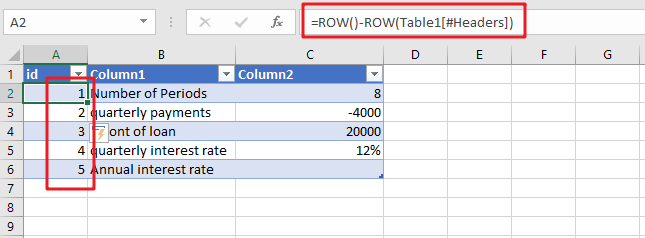When creating an Excel Table, you may use a calculation relying on the ROW function to insert row numbers automatically. The formula in A2, which has been copied down, is as follows:

`=ROW()-ROW(Table1[#Headers])`

Note: the default table name is Table1. And #Headers is the header in the Table1.

## Let’s See How This Formula Works:

When there is no parameter, the ROW function will return the row number for the current row. If A cell reference is supplied, and ROW function will retrieve the number of rows of the selected cell in question. When a range is specified, ROW returns the row number corresponding to the first row in the range.

For the above formula, there is no argument supplied to ROW, the first ROW function returns 2, located in cell A2. The second ROW function makes use of a structured reference, as follows:

`=ROW(Table1[#Headers])`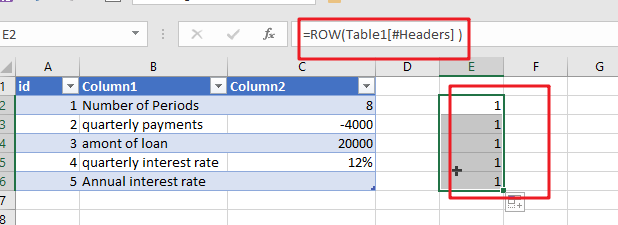The formula above works well as long as there is a header row in the table; however, the formula will fail if the heading row is disabled. If you are dealing with a table that does not have a header row, you may make use of the following method:

`=ROW()-INDEX(ROW(Table2),1,1)+1`

As in the previous formula, the initial ROW function will return the row that is currently being shown. Using the INDEX function, the first cell in the range Table2 (cell B2) is passed on to the second-ROW feature, which always returns the number 5. The following is how the formula works for the first three rows of the table: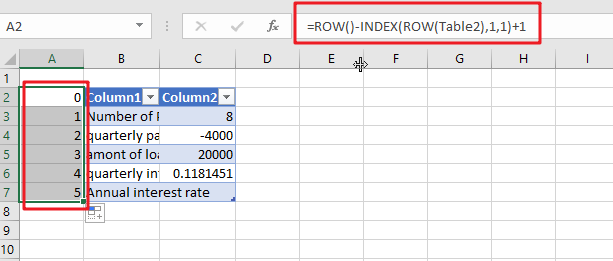Regardless of whether or not the header row is enabled, this equation will continue to operate correctly.

### Related Functions

• Excel INDEX function
The Excel INDEX function returns a value from a table based on the index (row number and column number)The INDEX function is a build-in function in Microsoft Excel and it is categorized as a Lookup and Reference Function.The syntax of the INDEX function is as below:= INDEX (array, row_num,[column_num])…
• Excel ROW function
The Excel ROW function returns the row number of a cell reference.The ROW function is a build-in function in Microsoft Excel and it is categorized as a Lookup and Reference Function.The syntax of the ROW function is as below:= ROW ([reference])….

## Get Address of First Cell in Range

We will learn how to get address of first cell in range in this post. At Instance, we must determine the address of first cell in range to use as the data table’s value. For the DATA table, we have a range of value values in the form of an array that is sent to the formula as input, and therefore the formula returns the first cell value.

The next sections include information on the functions mentioned before that we will use.

ADDRESS retrieves the address of a cell using the row and column numbers.

## Syntax:

`=ADDRESS(row_num, col_num, [abs_num] )`
• `Row_num`: The Row number
• `Col_num`: The Column number
• `[abs num]`: [Optional] integer, one of the following values: 1, 2, 3, or 4. If this parameter is ignored or set to 1, the returned address will be absolute, e.g. \$A\$1.

Now we’ll combine these functions into a formula. We’ll begin with a data table. We need to determine the data table’s initial cell address.

Utilize the following formula:

`=ADDRESS(ROW(table_array), COLUMN(table_array))`

Variables:

• `table array`: array representation of a data table

## Examples:

Let us validate this formula by applying it to an example.

Here we have a data table and we need to use the formula to get the first cell address in the same data table.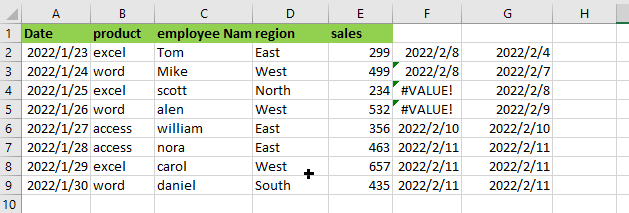Use the following Formula:

`= ADDRESS(ROW(A1:E9), COLUMN(A1:E9))`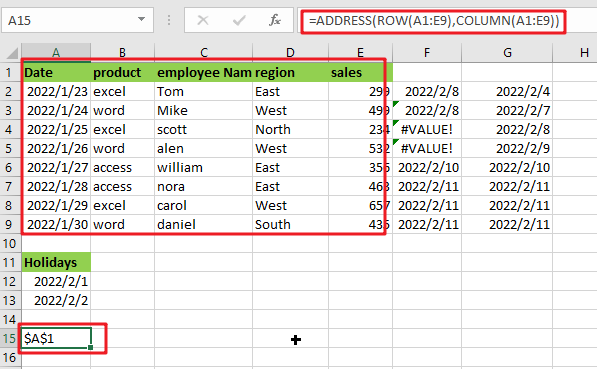Note: A1:E9 is array of tables

## Let’s See How This Formula Works:

`= COLUMN(A1:E9)`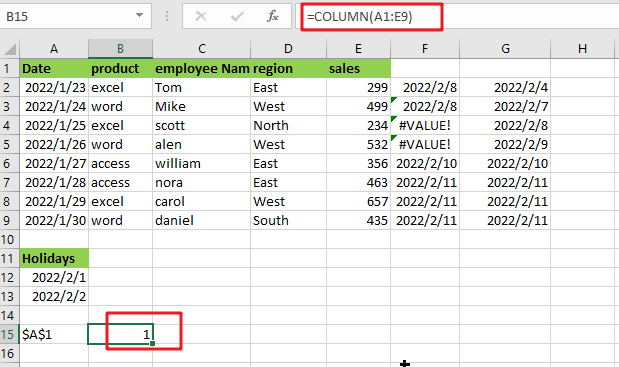The COLUMN function returns the value of the first column in the table array.

`= ROW(A1:E9)`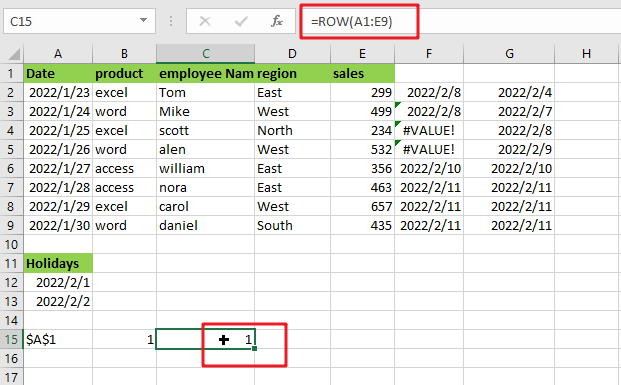The ROW function returns the index of the first row in the table array.

The ADDRESS function accepts the row number and column value parameters and returns the row’s and column’s absolute references.

The array sent to the function is denoted by cell reference. To get the result, press Enter.

As you can see, the formula works correctly since it delivers the value for the first cell in the data table.

Alternatively, you may use the CELL function:

CELL is an Excel built-in information function. Excel’s Cell function saves all the data in a specified cell and returns the cell’s info type. Using the follow formula:

`=CELL("address", [array_reference])`

or

`=CELL("address",INDEX(A1:E9,1,1))`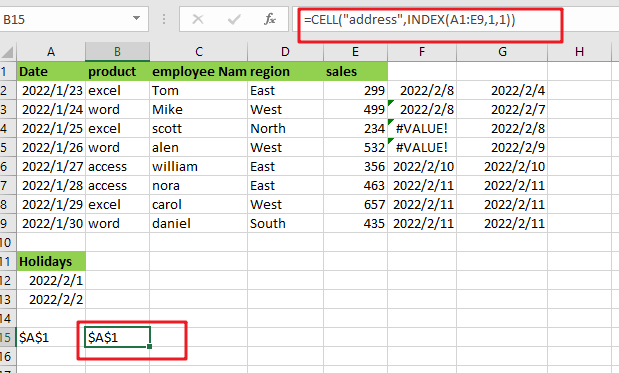Note:

• `[array_reference] `: table array given as reference.

As seen by the above calculation, you may use the first cell value as a reference.

The INDEX function can be used to get cell reference to the first cell in the given range by giving INDEX 1 for row number and 1 for column number.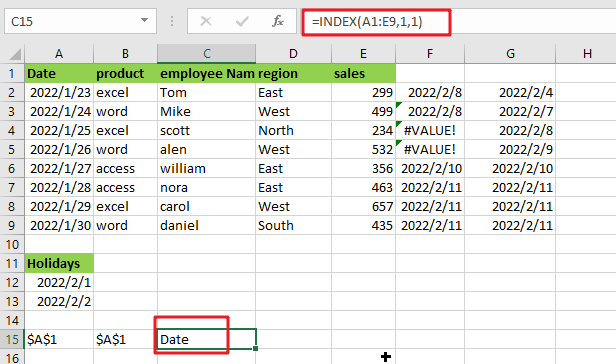The ADDRESS function may be used in conjunction with the ROW, COLUMN, and MIN functions to get the address of the first cell in a range. I hope this post on finding the get address of first cell in range in Excel was informative. It’s extremely easy and very helpful for people who use excel regularly.

### Related Functions

• Excel INDEX function
The Excel INDEX function returns a value from a table based on the index (row number and column number)The INDEX function is a build-in function in Microsoft Excel and it is categorized as a Lookup and Reference Function.The syntax of the INDEX function is as below:= INDEX (array, row_num,[column_num])…
• Excel ROW function
The Excel ROW function returns the row number of a cell reference.The ROW function is a build-in function in Microsoft Excel and it is categorized as a Lookup and Reference Function.The syntax of the ROW function is as below:= ROW ([reference])….
The Excel ADDRESS function returns a reference as a text string to a single cell.The syntax of the ADDRESS function is as below:=ADDRESS (row_num, column_num, [abs_num], [a1], [sheet_text])….
• Excel COLUMN function
The Excel COLUMN function returns the first column number of the given cell reference.The syntax of the COLUMN function is as below:=COLUMN ([reference])….
• Excel CELL function
The Excel CELL function returns information about the formatting, location, size, or contents of a cell.The syntax of the CELL function is as below:= CELL (info_type,[reference])…
• Excel MIN function
The Excel MIN function returns the smallest numeric value from the numbers that you provided. Or returns the smallest value in the array.The MIN function is a build-in function in Microsoft Excel and it is categorized as a Statistical Function.The syntax of the MIN function is as below:= MIN(num1,[num2,…numn])….

## Abbreviate Names Or Words in Excel

As an MS Excel user, you might have come across a task where you need to abbreviate different names or words, and there are also possibilities that you might have done this task manually by assuming that there isn’t any other way to do this task, but you assumed wrong because fortunately there is a way which would let you abbreviate names and words in a matter of seconds unlike doing it manually which consumes a lot of time and leaves you with no satisfying results.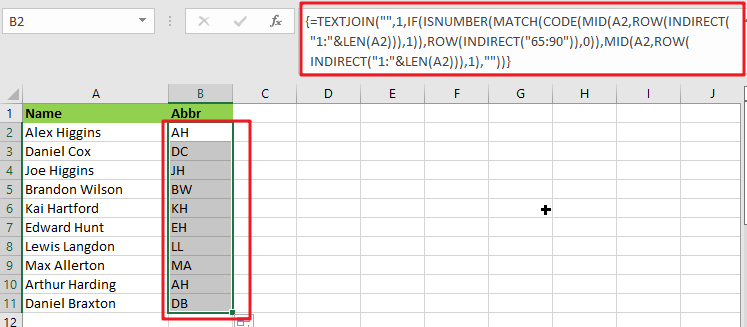So for exploring that way/method, let’s dive into the article.

## General Formula:

The array formula to abbreviate different names and words in few seconds is mentionedas follows:

`{=TEXTJOIN("",1,IF(ISNUMBER(MATCH(CODE(MID(A2,ROW(INDIRECT("1:"&LEN(A2))),1)),ROW(INDIRECT("65:90")),0)),MID(A2,ROW(INDIRECT("1:"&LEN(A2))),1),""))}`## Syntax Explanation:

To understand the working of the above formula, we first need to know about each syntax and how they contribute to abbreviating names and words in seconds.

• `TEXTJOIN`: The TEXTJOIN function in Microsoft Excel allows you to join two or more strings together, with each value separated by a delimiter. The TEXTJOIN function is an Excel built-in function classified as a String/Text Function.
• `IF`: The IF function is one of Excel’s most used functions, allowing you to create logical comparisons between a number and what you anticipate.
• `ISNUMBER`: When a cell contains a number, the ISNUMBER function returns TRUE; otherwise, it returns FALSE. ISNUMBER can be used to verify that a cell contains a numeric value or that the output of another function is a number.
• `MATCH`: The MATCH is a function used to find a lookup value in a row, column, or table in MS Excel. MATCH allows for approximate and accurate matching and wildcards (*?) for partial matches.
• `CODE`: The CODE function in Excel returns a numeric code for a specified character.
• INDIRECT: The INDIRECT function returns a range reference. This function may generate a reference that will not change if a row or column is added to the worksheet.

## Let’s See How This Formula Works:

To abbreviate capital letters text, use this array formula based on the TEXTJOIN function, new in Office 365 and Excel 2019. You may use this method to generate initials from names or acronyms. Because only capital letters can survive this algorithm, the original text must contain capitalized terms. If necessary, you can capitalize words using the PROPER function.

The formula in B2 in the case provided is:

`=TEXTJOIN("",1,IF(ISNUMBER(MATCH(CODE(MID(A2,ROW(INDIRECT("1:"&LEN(A2))),1)),ROW(INDIRECT("65:90")),0)),MID(A2,ROW(INDIRECT("1:"&LEN(A2))),1),""))`

The MID function is used to turn the string into an array of individual letters from the inside out:

`=MID(A2,ROW(INDIRECT("1:"&LEN(A2))),1)`

In this formula section, the operators MID, ROW, INDIRECT, and LEN transform a string into an array of letters.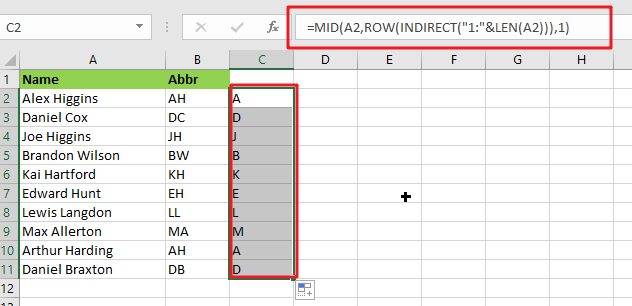MID delivers an array of all characters in the text.

`=CODE(MID(A2,ROW(INDIRECT("1:"&LEN(A2))),1))`

This above array is sent to the CODE function, which returns an array of numeric ASCII codes, one for each letter.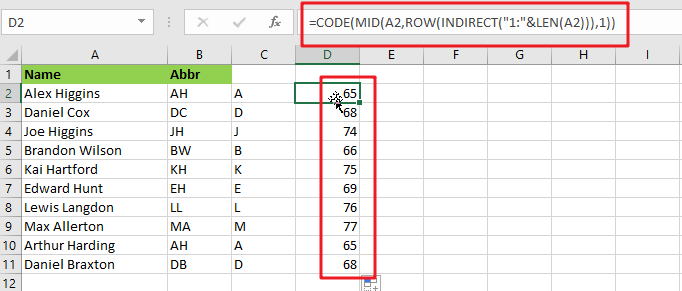`=ROW(INDIRECT("65:90")`

ROW and INDIRECT are used to generate another numeric array:

`{65;66;67;68;69;70;71;72;73;74;75;76;77;78;79;80;81;82;83;84;85;86;87;88;89;90}`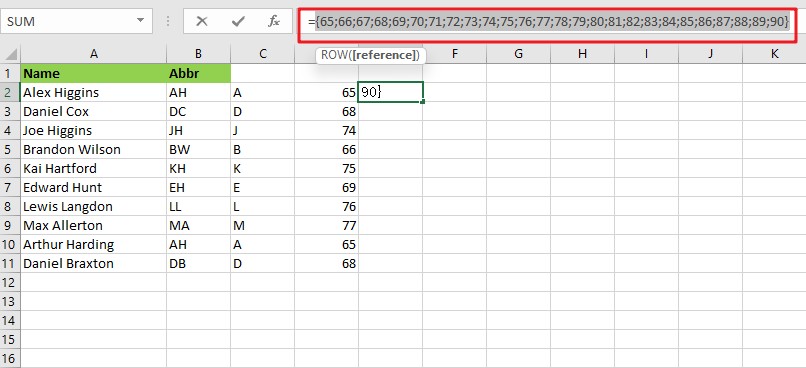Note: The digits 65 to 90 correspond to the ASCII codes for all capital letters from A to Z. This array is used as the lookup array in the MATCH function, and the original array of ASCII codes is given as the lookup value.

`{=MATCH(CODE(MID(A2,ROW(INDIRECT("1:"&LEN(A2))),1)),ROW(INDIRECT("65:90")),0)}`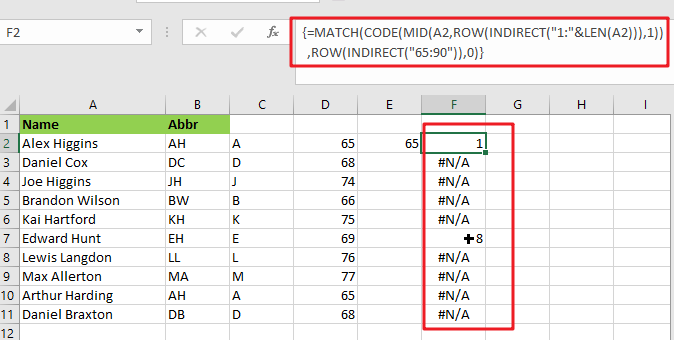The MATCH function returns either a number or the #N/A error. Because numbers represent capital letters.

`{=IF(ISNUMBER(MATCH(CODE(MID(A2,ROW(INDIRECT("1:"&LEN(A2))),1)),ROW(INDIRECT("65:90")),0)),MID(A2,ROW(INDIRECT("1:"&LEN(A2))),1),"")}`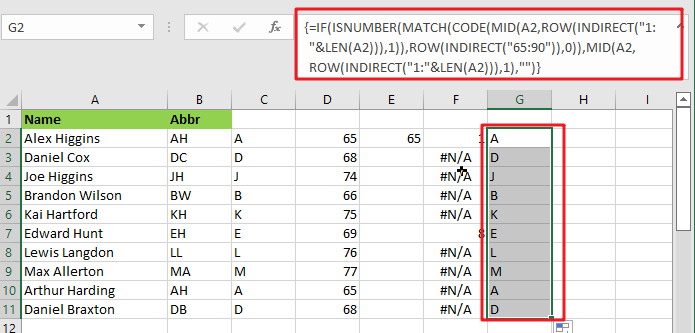The ISNUMBER function is used with the IF function to filter results. Only characters with ASCII codes between 65 and 90 will be included in the final array, reconstructed using the TEXTJOIN method to produce the final abbreviation or acronym.

## Abbreviate Names And Words In MS Excel 2016 Or Older Versions

As in Excel 2016 and earlier versions, the formula discussed above would not work, so the TRIM function is available in Excel 2016 and earlier versions, so we use it to abbreviate names and words.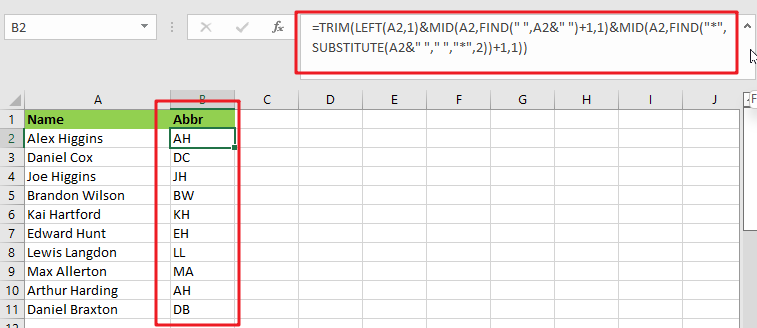### General Formula:

The formula we would use in MS Excel 2016 and earlier versions to abbreviate names and words as follows.

`=TRIM(LEFT(Text,1)&MID(Text,FIND(" ",Text&" ")+1,1)&MID(Text,FIND("*",SUBSTITUTE(Text&" ","*,"2))+1,1)`

### How Does This Formula Work?

From cell A2, if you want to extract the initials, enter this formula in cell B2.

`=TRIM(LEFT(A2,1)&MID(A2,FIND(" ",A2&" ")+1,1)&MID(A2,FIND("*",SUBSTITUTE(A2&" "," ","*",2))+1,1))`

Here the text string is the string you want to extract the first letters of each word.

When you press the Enter key, all of the first letters of each word in cell A2 are retrieved.### Explanation:

1. The TRIM function eliminates any extra spaces from the text string.
2. The LEFT(A2,1) function retrieves the first letter of the text string.
3. MID(A2,FIND(” “,A2&” “)+1,1) retrieves the initial letter of the second word separated by a space.
4. MID(A2,FIND(“*”,SUBSTITUTE(A2&” “,” “,”*,”2))+1,1) retrieves the initial letter of the third word separated by a space.

NOTE:

This formula only works when three or fewer words are in a cell. You can modify ” “ in the formula to different delimiters.

This formula extracts the initial characters in a case-insensitive manner; if you want the formula to always return in the upper case, include the UPPER function in the formula.

`=UPPER(TRIM(LEFT(A2,1)&MID(A2,FIND(" ",A2&" ")+1,1)&MID(A2,FIND("*",SUBSTITUTE(A2&" "," ","*",2))+1,1)))`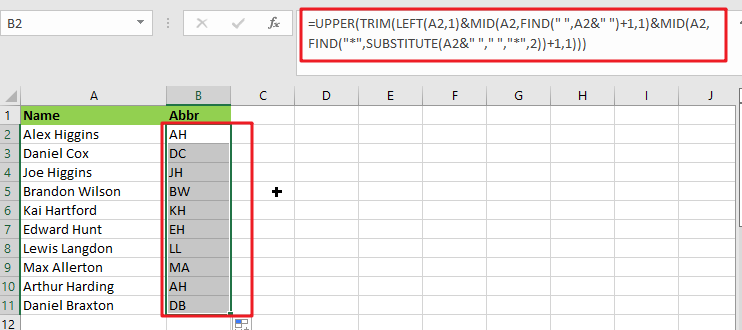### Related Functions

• Excel IF function
The Excel IF function perform a logical test to return one value if the condition is TRUE and return another value if the condition is FALSE. The IF function is a build-in function in Microsoft Excel and it is categorized as a Logical Function.The syntax of the IF function is as below:= IF (condition, [true_value], [false_value])….
• Excel TEXTJOIN function
The Excel TEXTJOIN function joins two or more text strings together and separated by a delimiter. you can select an entire range of cell references to be combined in excel 2016.The syntax of the TEXTJOIN function is as below:= TEXTJOIN  (delimiter, ignore_empty,text1,[text2])…
• Excel ROW function
The Excel ROW function returns the row number of a cell reference.The ROW function is a build-in function in Microsoft Excel and it is categorized as a Lookup and Reference Function.The syntax of the ROW function is as below:= ROW ([reference])….
• Excel MID function
The Excel MID function returns a substring from a text string at the position that you specify.The syntax of the MID function is as below:= MID (text, start_num, num_chars)…
• Excel INDIRECT function
The Excel INDIRECT function returns the cell reference based on a text string, such as: type the text string “A2” in B1 cell, it just a text string, so you can use INDIRECT function to convert text string as cell reference….
• Excel MATCH  function
The Excel MATCH function search a value in an array and returns the position of that item.The MATCH function is a build-in function in Microsoft Excel and it is categorized as a Lookup and Reference Function.The syntax of the MATCH function is as below:= MATCH  (lookup_value, lookup_array, [match_type])….
• Excel ISNUMBER function
The Excel ISNUMBER function returns TRUE if the value in a cell is a numeric value, otherwise it will return FALSE.The syntax of the ISNUMBER function is as below:= ISNUMBER (value)…
• Excel CODE function
The Excel CODE function returns the numeric ASCII value for the first character of a text string.The syntax of the CODE function is as below:= CODE  (text)…
• Excel LEN function
The Excel LEN function returns the length of a text string (the number of characters in a text string).The LEN function is a build-in function in Microsoft Excel and it is categorized as a Text Function.The syntax of the LEN function is as below:= LEN(text)…
• Excel LEFT function
The Excel LEFT function returns a substring (a specified number of the characters) from a text string, starting from the leftmost character.The LEFT function is a build-in function in Microsoft Excel and it is categorized as a Text Function.The syntax of the LEFT function is as below:= LEFT(text,[num_chars])…t)…
• Excel FIND function
The Excel FIND function returns the position of the first text string (sub string) within another text string.The syntax of the FIND function is as below:= FIND(find_text, within_text,[start_num])…
• Excel TRIM function
The Excel TRIM function removes all spaces from text string except for single spaces between words.  You can use the TRIM function to remove extra spaces between words in a string.The syntax of the TRIM function is as below:= TRIM (text)….

## Filter or Extract with a Partial Match

Assume you have a table consisting of a few cells having few values, and you want to filter out the set of records with the partial match. You might take it easy and would prefer to manually filter out the desired partial matching values into another table without any need for the formula; then congratulations because you are thinking right.

But let me add that it would be a big deal while dealing with a bulk of data in the table, and then doing this bulky task manually would be a foolish decision.

But there isn’t any need to worry about it because after carefully reading this article filtering out the set of records with the aid of partial matches will be a piece of cake for you.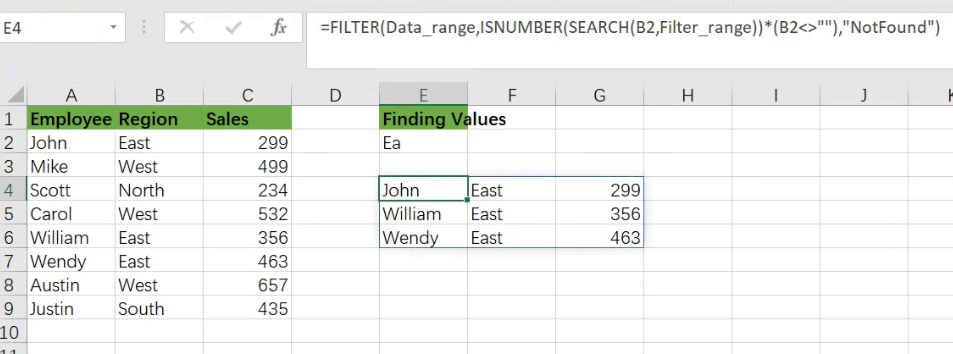So let’s get straight into it!

## General Formula:

You can use the FILTER function in combination with the SEARCH function to choose data records based on a partial match. The formula in E4 is written as follows:

`=FILTER(Data_range,ISNUMBER(SEARCH(B2,Filter_range))*(B2<>""),"Not Found")`

Note: Data_range is name range for A2:C9, and Filter_range is antoehr name range for B2:B9.## Let’s See How This Formula Works

The motive is to extract a collection of records that match a partial text string in this example. We match one column in the data range A2:C9 or the “Region” column. The FILTER function (new in Excel 365) retrieves matched data from a range based on a logical filter, which is at the heart of this formula:

`=FILTER(filter_data,filter_logic)`

The task in this example is to build the logic required to match records based on a partial match. Because the FILTER function does not handle wildcards, we must use an alternative technique. In this situation, we use the SEARCH function in conjunction with the ISNUMBER function as follows:

`=ISNUMBER(SEARCH(B2,Filter_range))`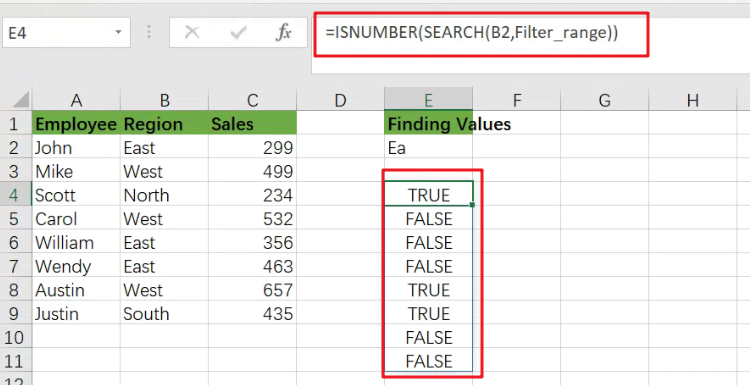The SEARCH function seeks text input in cell E2 within the Filter_range name range. SEARCH returns the position of a result in the text if it finds one.

If SEARCH formula does not yield any results, it returns the `#VALUE!` error:

We have a match if SEARCH returns a number. Otherwise, we don’t have a match. We wrap the SEARCH function within the ISNUMBER function to transform this result into a simple TRUE/FALSE value. Only when SEARCH returns a number will ISNUMBER return TRUE.

We aren’t utilizing a wildcard like (“*”) to achieve a partial match, but the SEARCH + ISNUMBER combination acts similarly. SEARCH function will return a number if the search string is found anywhere in the text, and ISNUMBER will return TRUE if the search string is found anywhere in the text.

`=FILTER(Data_range,ISNUMBER(SEARCH(B2,Filter_range)))`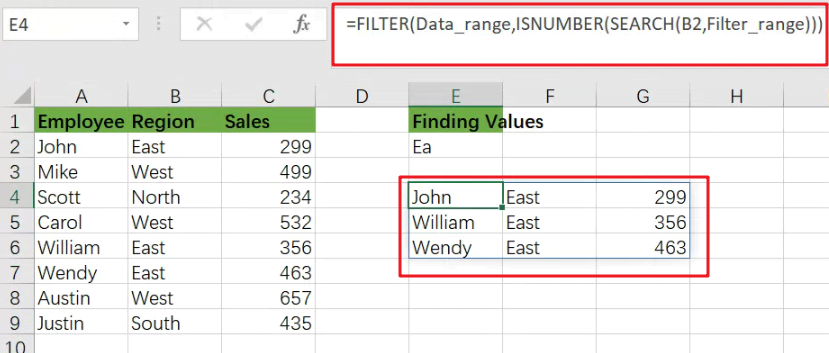We now have a workable formula, but we still need to clean up a few things. First, if the FILTER function returns no results, it will produce a `#CALC!` error. We would add a text message for the “if_empty” argument to deliver a friendlier message:

`=FILTER(Data_range,ISNUMBER(SEARCH(B2,Filter_range))*(B2<>""),"Not Found")`

Finally, we must deal with the circumstance where the search string in E2 is blank. Surprisingly, if the search text is an empty string, the SEARCH function will return the value 1.

If field B2 is empty, FILTER will return all results since ISNUMBER will joyfully return TRUE for number 1. To avoid this behavior, we add the following logic to the original logical expression:

`=ISNUMBER(SEARCH(B2,Filter_range))*(B2<>"")`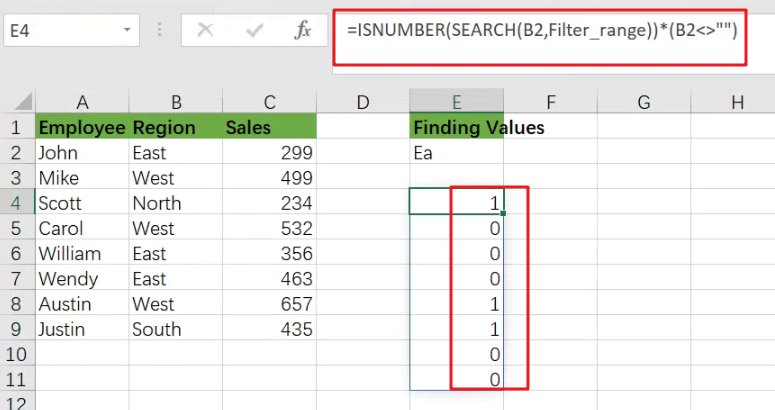When B2 is not empty, the expression B2<>”” yields TRUE; otherwise, it returns FALSE. By the original SEARCH + ISNUMBER expression, when we would multiply the results of this expression, all TRUE results are “canceled out” when B2 is empty. This is a variation on Boolean logic.

## Extract All Partial Match Using Index and Match function

Only Excel 365 supports the FILTER feature. It is feasible to put up a partial match formula in previous versions of Excel to produce more than one match, but it is more complicated. This following formula demonstrates one method based on INDEX and MATCH.

`=INDEX(\$B\$1:\$B\$5,AGGREGATE(15,6,(ROW(\$B\$1:\$B\$5)-ROW(\$B\$1)+1)/ISNUMBER(SEARCH(\$D\$1,\$B\$1:\$B\$5)),E2))`

### Related Functions

• Excel INDEX function
The Excel INDEX function returns a value from a table based on the index (row number and column number)The INDEX function is a build-in function in Microsoft Excel and it is categorized as a Lookup and Reference Function.The syntax of the INDEX function is as below:= INDEX (array, row_num,[column_num])…
• Excel ROW function
The Excel ROW function returns the row number of a cell reference.The ROW function is a build-in function in Microsoft Excel and it is categorized as a Lookup and Reference Function.The syntax of the ROW function is as below:= ROW ([reference])….
• Excel SMALL function
The Excel SMALL function returns the smallest numeric value from the numbers that you provided. Or returns the smallest value in the array.The syntax of the SMALL function is as below:=SMALL(array,nth) …
• Excel IF function
The Excel IF function perform a logical test to return one value if the condition is TRUE and return another value if the condition is FALSE. The IF function is a build-in function in Microsoft Excel and it is categorized as a Logical Function.The syntax of the IF function is as below:= IF (condition, [true_value], [false_value])….
• Excel ISNUMBER function
The Excel ISNUMBER function returns TRUE if the value in a cell is a numeric value, otherwise it will return FALSE.The syntax of the ISNUMBER function is as below:= ISNUMBER (value)…
• Excel SEARCH function
The Excel SEARCH function returns the number of the starting location of a substring in a text string.The syntax of the SEARCH function is as below:= SEARCH  (find_text, within_text,[start_num])…
• Excel AGGREGATE function
The Excel AGGREGATE function returns an aggregate in a list or database and ignore errors or hidden rows. The syntax of the AGGREGATE function is as below:= AGGREGATE(function_num, options, ref1,[ref2])…
• Excel Filter function
The FILTER function extracts matched records from a collection of data using one or more logical checks. The include argument specifies logical tests, which might encompass a wide variety of formula conditions.==FILTER(array,include,[if empty])…

## Extract matching values From Two Lists

Suppose that you are working with two lists containing few values, and you want to extract the matching values from those two lists into another separate list. You might prefer to manually extract the matching values from the two lists, which is ok for the few values, but it would be a big deal to extract the matching values from the two lists having multiple cells, and doing it manually would be a foolish attempt because there are 90% chances that you would 100% get tired of it and would never complete your work on time.

But don’t be worry about it because after carefully reading this article, extracting the matching values from the two lists into another separate list would become a piece of cake for you.

So let’s dive into the article to take you out of this fix.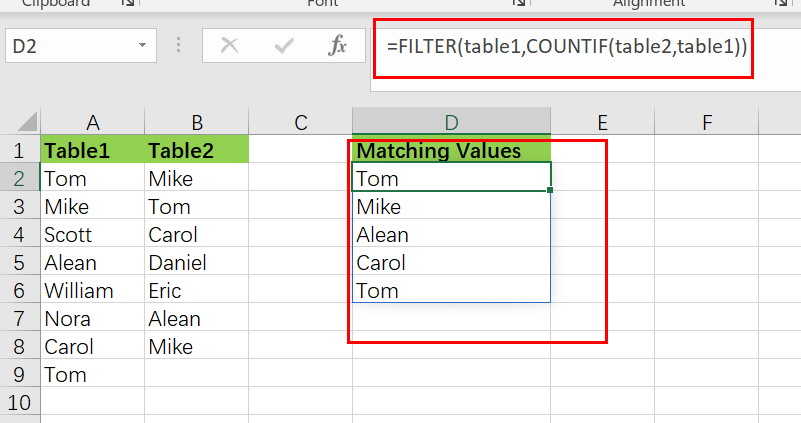## General Formula

The Following Formula would help you compare and extract the matching values from the two lists into another separate list.

`=FILTER(table1,COUNTIF(table2,table1))`

This Formula is based on the FILTER and COUNTIF functions, where the `table1(A2:A9)` and `table2 (B2:B8)` are the named ranges, the list in the `range (D2:D6)` is the list containing the matching values by comparing both table1 and table2.

## Syntax Explanations

Before going into the explanation of the Formula for getting the work done efficiently, we must understand each syntax which would make it easy for you that how each syntax contributes to executing the matching values from the two lists into another separate list:

• `Filter`: This function contributes to narrowing down or filtering out a range of data on the user-defined criteria.
• `COUNTIF`: It is a statistical function that counts the number of cells to meet specific criteria.
• `List`: In this Formula, the list represents the two lists present in the excel worksheet to execute the common values.
• `Comma symbol (,)`: In Excel, this comma symbol acts as a separator that helps to separate a list of values.
• `Parenthesis ()`: The core purpose of this Parenthesis symbol is to group the elements and separate them from the rest of the elements.

## Let’s See How This Formula Works

The FILTER function is used in this Formula to extract data based on a logical test created using the COUNTIF function:

`=FILTER(table1,COUNTIF(table2,table1))`The COUNTIF function is being used to generate the actual filter.

`=COUNTIF(table2,table1))`

It’s worth noticing that we’re using table2 as the range argument and table1 as the criterion argument. In other words, we’re asking COUNTIF to count all values in table1 that occur in table2. We obtain an array with several results since we provide COUNTIF various values for criteria:

`{1;2;0;1;0;0;0;1}`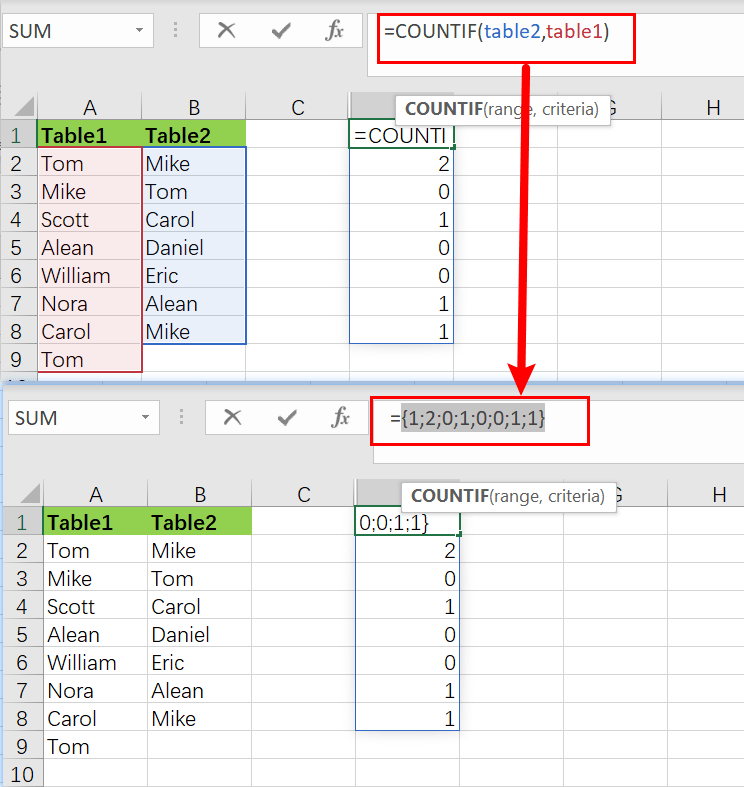Note that the array has 8 counts for each element in the table1. A zero value denotes a value in a table1 that is not present in the table2. Any other positive number denotes a value in table1 that is also present in table2. As the include parameter, this array is passed straight to the FILTER function:

`=FILTER(table1,{1;1;0;1;0;1;0;0;1;0;1;1})`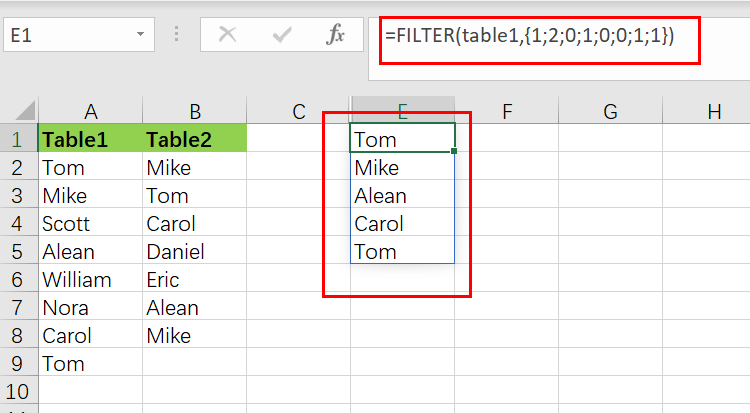The array is used as a filter by the filter function. Any item in the table1 connected with a zero would be eliminated, but any value related to a positive number would retain.

## Extract Non-matching Values

If you want to remove the non-matching values from table1, values in table1 that do not present in table2, we would need to modify the above formula, which is stated as follows:

`=FILTER(table1,NOT(COUNTIF(table2,table1)))`

The NOT function reverses the COUNTIF result, and every non-zero integer returns FALSE, and any zero value returns TRUE. The output is a list of all the values in the table1 that aren’t on the table2.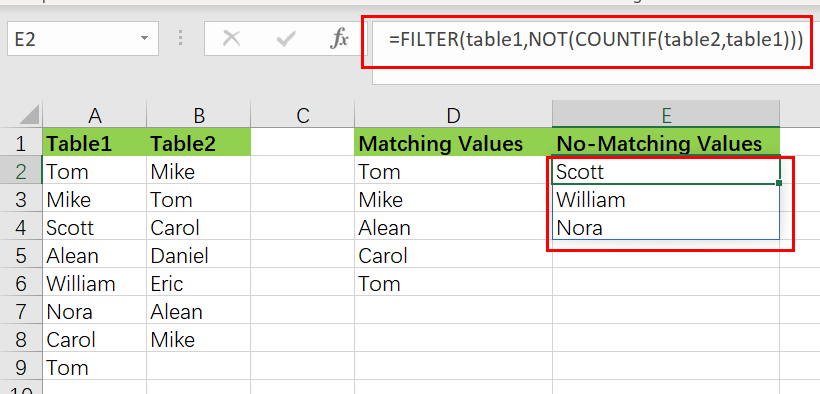## Method two: Extract Matching Values Using INDEX

You can also create a newly formula to extract matching values without the FILTER function, but the Formula would become more complicated. And the formula based on the INDEX function. Like below:

`=IFERROR(INDEX(table1,SMALL(IF(COUNTIF(table2,table1),ROW(table1)-ROW(INDEX(table1,1,1))+1),ROWS(\$D\$2:D2))),"")`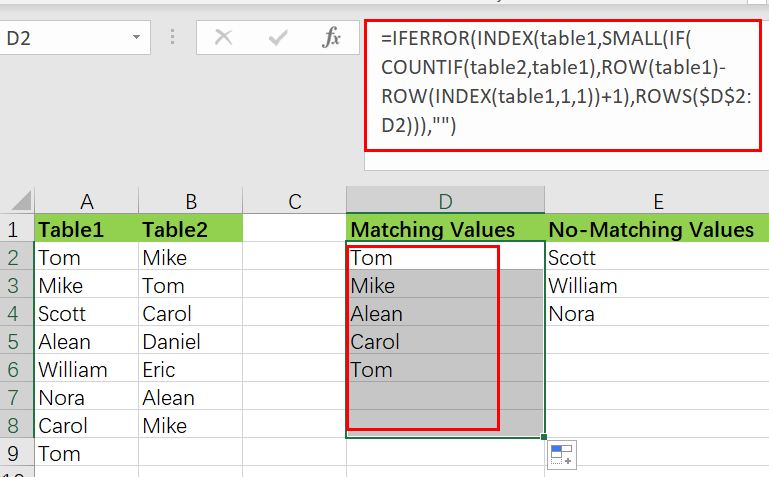Except in Excel 365, this array formula must be typed using `control + shift + enter`.

The INDEX function, which takes table1 as an array parameter, lies at the heart of this Formula. The majority of the remaining Formula determines the row number for matching values. This expression creates a list of relative row numbers as follows:

`=ROW(table1)-ROW(INDEX(table1,1,1)) +1`

which yields a 12-number array reflecting the rows in table1:

`{1;2;3;4;5;6;7;8;}`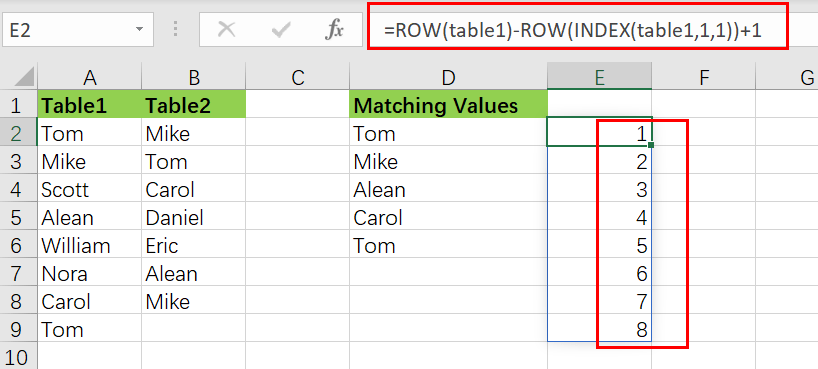These are filtered using the IF function and the same methodology used above in the FILTER Function, but this time using the COUNTIF function:

`=COUNTIF(table2,table1) / identify values that match`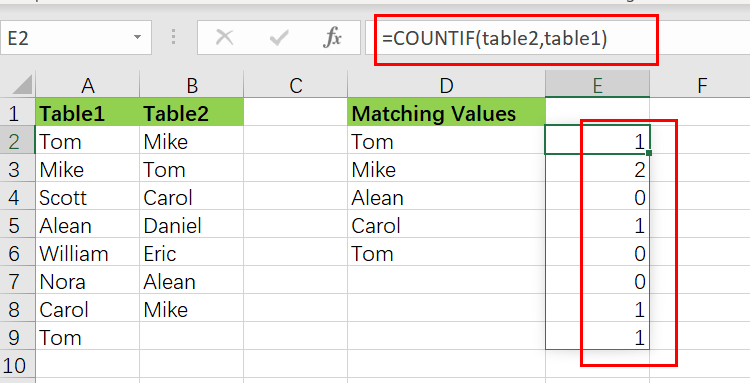`=IF(COUNTIF(table2,table1),ROW(table1)-ROW(INDEX(table1,1,1))+1)`

The resultant array is as follows:

`{1;2;FALSE;4;FALSE; FALSE;7;8; }`

As the Formula is copied along the column, this array is sent immediately to the SMALL function.

The IFERROR function is designed to catch mistakes when a formula is copied down, and the matching values run out.

### Related Functions

• Excel COUNTIF function
The Excel COUNTIF function will count the number of cells in a range that meet a given criteria. This function can be used to count the different kinds of cells with number, date, text values, blank, non-blanks, or containing specific characters.etc.= COUNTIF (range, criteria)…
• Excel Filter function
The FILTER function extracts matched records from a collection of data using one or more logical checks. The include argument specifies logical tests, which might encompass a wide variety of formula conditions.==FILTER(array,include,[if empty])…
• Excel INDEX function
The Excel INDEX function returns a value from a table based on the index (row number and column number)The INDEX function is a build-in function in Microsoft Excel and it is categorized as a Lookup and Reference Function.The syntax of the INDEX function is as below:= INDEX (array, row_num,[column_num])…
• Excel IF function
The Excel IF function perform a logical test to return one value if the condition is TRUE and return another value if the condition is FALSE. The IF function is a build-in function in Microsoft Excel and it is categorized as a Logical Function.The syntax of the IF function is as below:= IF (condition, [true_value], [false_value])….
• Excel ROW function
The Excel ROW function returns the row number of a cell reference.The ROW function is a build-in function in Microsoft Excel and it is categorized as a Lookup and Reference Function.The syntax of the ROW function is as below:= ROW ([reference])….
• Excel SMALL function
The Excel SMALL function returns the smallest numeric value from the numbers that you provided. Or returns the smallest value in the array.The syntax of the SMALL function is as below:=SMALL(array,nth) …
• Excel IFERROR function
The Excel IFERROR function returns an alternate value you specify if a formula results in an error, or returns the result of the formula.The syntax of the IFERROR function is as below:= IFERROR (value, value_if_error)….
• Excel NOT function
The Excel NOT function returns the opposite of a given logical or Boolean value. For example, if you supplied with the value TRUE, the NOT function will return FALSE; If you supplied with the value FALSE, and the NOT function will TRUE. The syntax of the NOT function is as below:=NOT(logical)…

## Extract all Partial Matches

Just assume that you have a range of data that contains a text string and you want to extract the few partial matches into another separate range of cells; then you might think that it’s not a big deal; because you would prefer to manually extract the few partial matches into another separate range of cells without any need of the formula;

Then congratulations because you are thinking right, but let me add up that it would be a big deal to extract the multiple partial matches into another separate range of cells and doing it manually would be a foolish attempt because you would get tired of it and would never complete your work on time.

But don’t be worry about it because after carefully reading this article, extracting multiple partial matches into another range of cells would become a piece of cake for you.

So let’s dive into the article to take you out of this fix.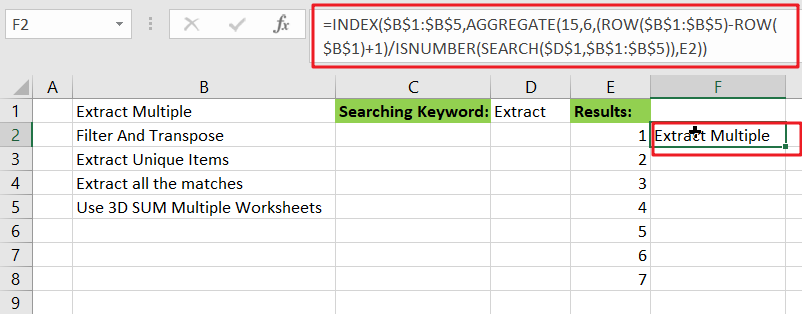## General formula

The Following formula would help you out for extracting multiple partial matches into another separate range of cells:

`=INDEX(\$B\$1:\$B\$5,AGGREGATE(15,6,(ROW(\$B\$1:\$B\$5)-ROW(\$B\$1)+1)/ISNUMBER(SEARCH(\$D\$1,\$B\$1:\$B\$5)),E2))`

## Syntax Explanations

Before going into the explanation of the formula for getting the work done efficiently, we must understand each syntax which would make it easy for you that how each syntax contributes to extracting multiple partial matches into a separate range or list of cells:

• `INDEX`: In a range or array, this index function contributes to returning the value at a given position.
• `AGGREGATE`: This function contributes to returning the aggregate result in a database or list of values in the excel sheet.
• `ROW`: In Excel, this Row function contributes toreturning the row number as a reference.
• `Absolute Reference`: The Absolute referenceis nothing but an actual fixed location in a worksheet.
• `ISNUMBER`: This function contributes to returns TRUE when a cell contains a number and FALSE if there is no number.
• `SEARCH`: This function contributes to locating the character between two text strings and returns to the number of the starting position of the first text string from the first character of the second text string.
• `Comma symbol` (,): In Excel, this comma symbol acts as a separator that helps to separate a list of values.
• `Minus Operator` (-): This minus symbol contributes to subtracting any two values.
• `Parenthesis` (): The core purpose of this Parenthesis symbol is to group the elements and to separate them from the rest of the elements.
• `Plus operator` (+): This plus operator adds the values.
• `Division` (/): This division symbol is used for dividing values or numbers.

## Let’s See How This Formula Works:

The INDEX function is the primary function having AGGREGATE, which is highly useful to figure out the matches for each row in the extracted area:

`=INDEX(\$B\$1:\$B\$5,matched_values)`

Almost all of the work is to determine and report which rows in “`\$B\$1:\$B\$5`” match the search string and report the position of each matching value to INDEX. This is accomplished by configuring the AGGREGATE function as follows:

`=AGGREGATE(15,6,(ROW(\$B\$1:\$B\$5)-ROW(\$B\$1)+1)/ISNUMBER(SEARCH(\$D\$1,\$B\$1:\$B\$5)),E2)`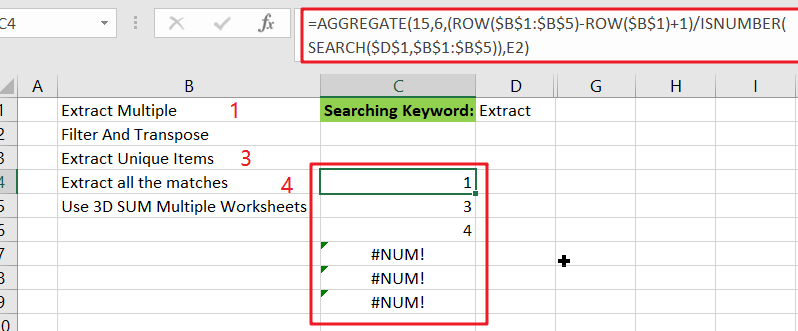The very first input or argument, which is `15`, instructs AGGREGATE to return the nth smallest values.

The second argument, `6`, specifies whether or not to ignore mistakes.

The third argument is an expression that returns an array of results that match.

The fourth input, `E2`, it specifies the “nth” value.

AGGREGATE works on arrays, and for the third argument inside AGGREGATE, the expression below builds an array :

`={(ROW(\$B\$1:\$B\$5)-ROW(\$B\$1)+1)/ISNUMBER(SEARCH(\$D\$1,\$B\$1:\$B\$5))}}`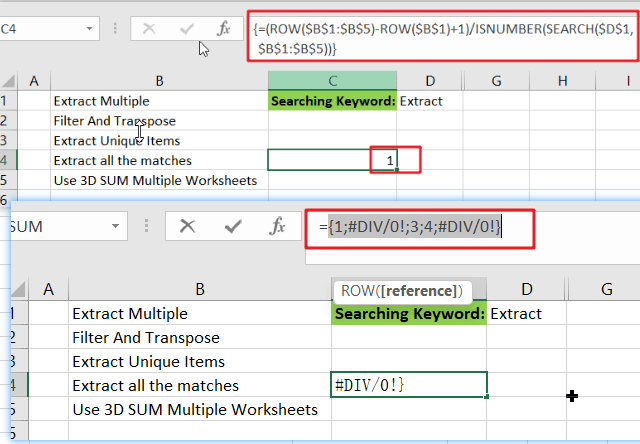In this case, the ROW function is used to build an array of relative row numbers, as that’s why SEARCH and ISNUMBER are combined to match the search string against values in the data, resulting in an array of TRUE and FALSE values.

TRUE behaves as 1 in this math operation, while FALSE behaves as 0. As a result, row numbers with a positive match are divided by 1 and survive the operation, whereas row numbers with non-matching values are destroyed and become `#DIV/0 `errors. AGGREGATE is configured to ignore errors; it ignores #DIV/0 errors and returns the “nth” smallest number from the remaining values.

## Alternative Formula with SMALL Function

You can also use an alternative formula which is based on SMALL function and IF Function to achieve the same result:

`=INDEX(\$B\$1:\$B\$5,SMALL(IF(ISNUMBER(SEARCH(\$D\$1, \$B\$1:\$B\$5)),ROW(\$B\$1:\$B\$5)-ROW(\$B\$1)+1),E2))`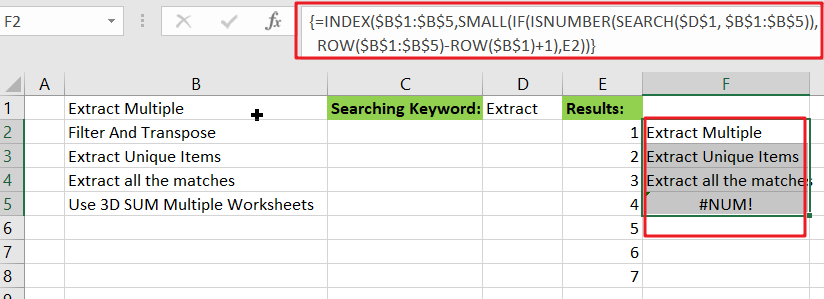Note: this is an array formula, and to get the work done, please enter it with `Control` `+` `Shift` `+` `Enter`.

### Related Functions

• Excel INDEX function
The Excel INDEX function returns a value from a table based on the index (row number and column number)The INDEX function is a build-in function in Microsoft Excel and it is categorized as a Lookup and Reference Function.The syntax of the INDEX function is as below:= INDEX (array, row_num,[column_num])…
• Excel ROW function
The Excel ROW function returns the row number of a cell reference.The ROW function is a build-in function in Microsoft Excel and it is categorized as a Lookup and Reference Function.The syntax of the ROW function is as below:= ROW ([reference])….
• Excel SMALL function
The Excel SMALL function returns the smallest numeric value from the numbers that you provided. Or returns the smallest value in the array.The syntax of the SMALL function is as below:=SMALL(array,nth) …
• Excel IF function
The Excel IF function perform a logical test to return one value if the condition is TRUE and return another value if the condition is FALSE. The IF function is a build-in function in Microsoft Excel and it is categorized as a Logical Function.The syntax of the IF function is as below:= IF (condition, [true_value], [false_value])….
• Excel ISNUMBER function
The Excel ISNUMBER function returns TRUE if the value in a cell is a numeric value, otherwise it will return FALSE.The syntax of the ISNUMBER function is as below:= ISNUMBER (value)…
• Excel SEARCH function
The Excel SEARCH function returns the number of the starting location of a substring in a text string.The syntax of the SEARCH function is as below:= SEARCH  (find_text, within_text,[start_num])…
• Excel AGGREGATE function
The Excel AGGREGATE function returns an aggregate in a list or database and ignore errors or hidden rows. The syntax of the AGGREGATE function is as below:= AGGREGATE(function_num, options, ref1,[ref2])…

## Extract Multiple Match Values into Separate Columns

If you have a few values/items in the excel sheet and you are thinking that with the aid of the “VlOOKUP” function you can look for a specific value, extract it and then put the matching item into the separate column in Ms Excel easily, then congratulations, you are thinking right, but here a problem arises that there isn’t any doubt that by this way you can extract one or two matches into the separate column easily but with the aid of this way you cannot extract multiple matches into separate columns and if you would do that by this way then there are 90% chances that you would 100% get tired of it and can’t complete your task at the right time.

But don’t be worry about it because after carefully reading this article extracting multiple matches into the separate columns would become a piece of cake for you.

So let’s dive into the article to take you out of this fix.

## General Formula:

For extracting multiple matches(items) into seprate columns you can use the  Array Formula which is based upon INDEX and SMALL, which is stated as follows:

`=IFERROR(INDEX(STU_Range,SMALL(IF(CLASS_Range=\$E2, ROW(STU_Range) -MIN (ROW(STU_Range))+1),COLUMNS(\$E\$2:E2))),"")`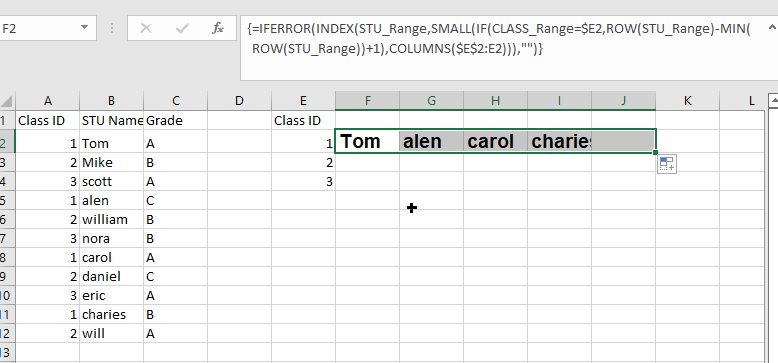## Syntax Explanations:

Before knowing about how to use this formula for getting the work done efficiently, we must understand each syntax which would make it easy for you that how each syntax contributes to extracting multiple matches into the separate columns:

• `IFERROR`: This Function returns a custom result whenever a formula generates an error and returns the expected result when no error is detected.
• `INDEX`: In a range or array, this index function contributes to returning the value at a given position.
• `SMALL`: From the given range of data, this small Function returns the Nth
• `IF`: In Excel, this IF Function contributes to returning two different values, one value for the TRUE result and another for the FALSE result.
• `ROW`: In Excel, this Row function contributes toreturning the row number as a reference.
• `MIN`: From the range of input values, this MIN function contributes to returning the smallest numeric value.
• `Absolute Reference`: The Absolute referenceis nothing but an actual fixed location in a worksheet.
• `COLUMNS`: From a given reference, this Column function contributes to counting the columns.
• `Comma symbol (,)`: This symbol acts as a separator that contributes to separating a list of values.
• `Minus Operator (-)`: This minus symbol contributes to subtracting any two values.
• `Parenthesis ()`: The primary purpose of this parenthesis symbol is to group the various elements.
• `Name`– It represents the input ranges in your worksheet.
• `Plus operator (+)`: This plus symbol adds the values.

## Let’s See How This Formula Works:

To use this array formula for getting the work done, you must enter this formula with Control + Shift + Enter. As soon as you would enter this formula into the first cell, you need to drag it down and across to fill in the other cells.

As you can see in the above screenshot, this formula uses two names ranging: “ CLASS_Range ” and “ STU_Range,” where “ STU_Range ” refers to B2:B12 and on the other hand “ CLASS_Range ” refers to A2:A12.

You would definitely wonder that how this formula works to extract multiple matches into columns? So here is the answer. In this formula, we use the Small Function and INDEX function, which work together.

As the SMALL Function (dynamically constructed by IF) is used to obtain row number corresponding to an “nth match,” so after getting the row number from SMALL Function, this would then pass it into the INDEX function, which returns the value at that row, this is also the main motive of this formula.

The snippet “IF(CLASS_Range=\$E2, ROW(STU_Range) -MIN (ROW(STU_Range))+1” tests the named range “ STU_Range ” for the value in E2. If the value is found, then from an array of relative row numbers, it would return a row number, which is created with:

`=ROW(STU_Range) -MIN (ROW(STU_Range))+1`

The output of this formula is :

`{1;2;3;4;5;6;7;8;9;10;11}`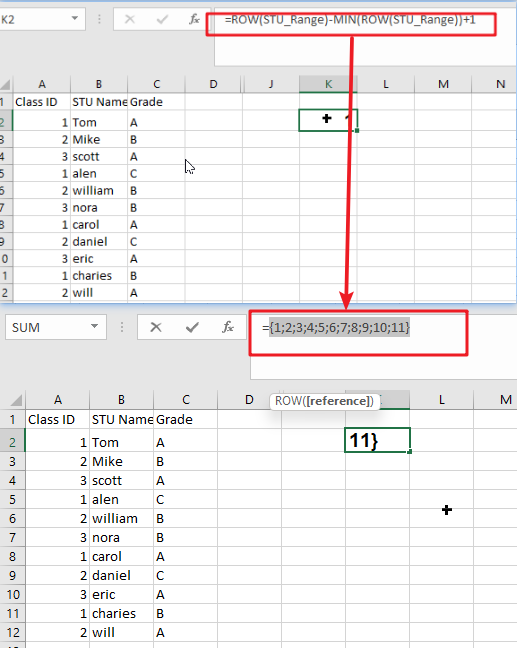Now the final result is an array that would contain the numbers where there is a match, and FALSE where there is not any match found:

`{1;FALSE;FALSE;4;FALSE;FALSE;7;FALSE;FALSE;10;FALSE}`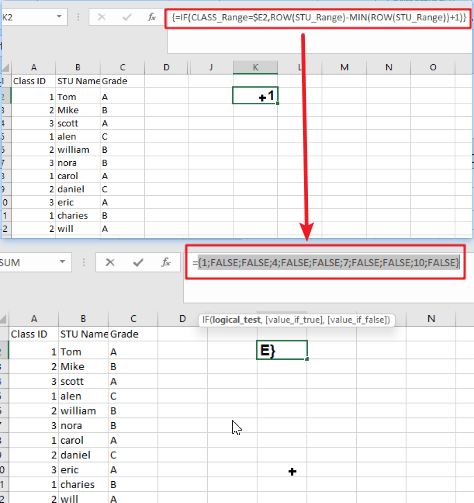Then this array goes into the SMALL Function. By expanding range(Given Below), The k value for SMALL (nth) returns:

`COLUMNS(\$E\$2:E2)`

The SMALL function returns each matching row number, which is then supplied as the row_num to the INDEX function as the array with the range named “ STU_Range.”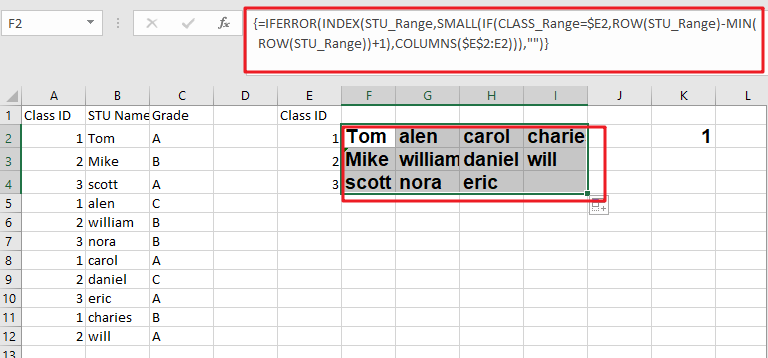Notes:

Now, this question would pop up in your mind that how would it handle the errors? Then whenever the COLUMN would return a value for k that does not exist, the #NUM error would be thrown by the SMALL Function at the next moment. This usually occurs when all the matches have occurred. To tackle the errors, the formula is wrapped up in the Function named “IFERROR,” which would receive the errors and then return an empty string (” “).

### Related Functions

• Excel INDEX function
The Excel INDEX function returns a value from a table based on the index (row number and column number)The INDEX function is a build-in function in Microsoft Excel and it is categorized as a Lookup and Reference Function.The syntax of the INDEX function is as below:= INDEX (array, row_num,[column_num])…
• Excel MATCH  function
The Excel MATCH function search a value in an array and returns the position of that item.The MATCH function is a build-in function in Microsoft Excel and it is categorized as a Lookup and Reference Function.The syntax of the MATCH function is as below:= MATCH  (lookup_value, lookup_array, [match_type])….
• Excel IF function
The Excel IF function perform a logical test to return one value if the condition is TRUE and return another value if the condition is FALSE. The IF function is a build-in function in Microsoft Excel and it is categorized as a Logical Function.The syntax of the IF function is as below:= IF (condition, [true_value], [false_value])….
• Excel ROW function
The Excel ROW function returns the row number of a cell reference.The ROW function is a build-in function in Microsoft Excel and it is categorized as a Lookup and Reference Function.The syntax of the ROW function is as below:= ROW ([reference])….
• Excel SMALL function
The Excel SMALL function returns the smallest numeric value from the numbers that you provided. Or returns the smallest value in the array.The syntax of the SMALL function is as below:=SMALL(array,nth) …
• Excel MIN function
The Excel MIN function returns the smallest numeric value from the numbers that you provided. Or returns the smallest value in the array.The MIN function is a build-in function in Microsoft Excel and it is categorized as a Statistical Function.The syntax of the MIN function is as below:= MIN(num1,[num2,…numn])….
• Excel IFERROR function
The Excel IFERROR function returns an alternate value you specify if a formula results in an error, or returns the result of the formula.The syntax of the IFERROR function is as below:= IFERROR (value, value_if_error)….
• Excel COLUMN function
The Excel COLUMN function returns the first column number of the given cell reference.The syntax of the COLUMN function is as below:=COLUMN ([reference])….

## Average the Last N Numeric Values in Excel

AVERAGE function is one of the most popular functions in Excel. Apply AVERAGE together with some other functions, we can calculate average simply for some complex situations.

In this article, we will introduce you to calculate average of the last N numbers from a range contains both numeric values and non-numeric values.

## EXAMPLE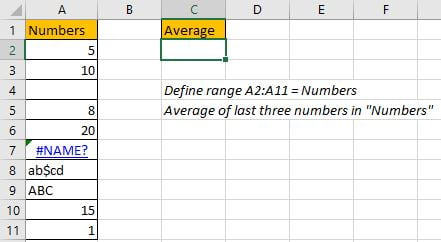In this case, we want to calculate the average of the last 3 numeric values in “Numbers” column. As non-numeric value “ABC” exists in last three cells, so we cannot directly apply AVERAGE(A9:A11) directly. To calculate average ignoring invalid values, we need the help of other functions.

In this article, to approach our goal, except the main AVERAGE function, we also apply ROW, ISNUMBER, IF, LARGE, LOOKUP functions.

## SOLUTION

To create a formula to get average in this case, we need to know:

1) Distinguish numbers and non-numeric values from range “Numbers”. Ignore non-numeric values in calculation.

2) Find out the last three cells with numbers in “Numbers”.

3) Find out last three values through searching for the last three positions.

## FORMULA with AVERAGE & OTHER FUNCTIONS

In C2, we input the formula

`=AVERAGE(LOOKUP(LARGE(IF(ISNUMBER(Numbers),ROW(Numbers)),{1,2,3}), ROW(Numbers), Numbers)).`

After typing, press Enter, average of the last three numbers is 12.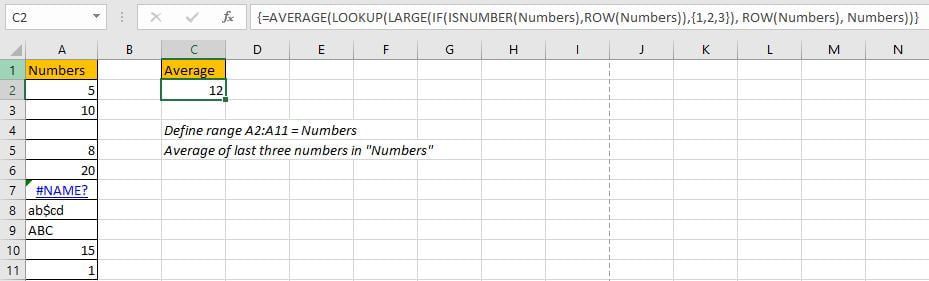In “Numbers” list, the last three values are 20, 15 and 1, the average is (20+15+1)/3=12, so the formula returned value is correct. The last three values are found out properly.

## FUNCTION INTRODUCTION

The main function in this formula is “AVERAGE”, it can return the average of last three values; Others are supported to find out numbers (ignoring non-numeric values), mark row numbers for the last three numbers, and the through searching row numbers to return corresponding numbers.

1. ROW function returns row number for a given range reference.

Syntax:

`=ROW(reference)`

Example. ROW(A1:A3) returns row numbers of range A1:A3, so we get {1;2;3}.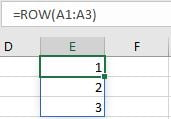2. ISNUMBER function returns True (for numeric values) or False (for strings, errors) based on value is a numeric value or not. For blank cells, it returns False.

Syntax:

`=ISNUMBER(value)`

Example. You can input a value, a cell reference, or a range reference for “value”.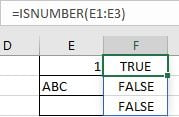3. IF function returns “true value” or “false value” based on the result of provided logical comparison. It is one of the most popular function in Excel.

Syntax:

`=IF(logical_test,[value_if_true],[value_if_false])`

Example, =IF(ISNUMBER(E1:E3),”Yes”,”No”).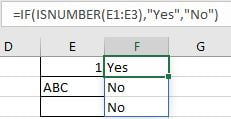4. LARGE function returns the Kth largest number from a given set of numbers or a range reference.

Syntax:

`=LARGE(array,k)`

Example, =LARGE(E1:E3,{1;2}), an array {3;2} is returned. If you set k=2, only 2 is returned.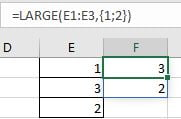5. LOOKUP function can through searching for a row or column to return the corresponding value in the same position but in the second row or column. It has two different syntaxes, in this case, it is for vector:

Syntax:

`=LOOKUP(lookup_value, lookup_vector, [result_vector])`

Example, =LOOKUP(H2,E2:E3,F2:F3), apply lookup to find out name for id1.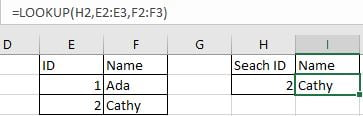6. AVERAGE function returns the average of numbers from a given range reference.

Syntax:

`=AVERAGE(number1, [number2], …)`

Example. For arguments “number1, number2,…”, they can be a set of numbers, or an array of numbers like {1;2;3}.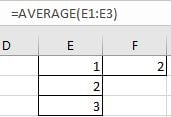## FORMULA EXPLANATION

`=AVERAGE(LOOKUP(LARGE(IF(ISNUMBER(Numbers),ROW(Numbers)),{1,2,3}), ROW(Numbers), Numbers))`

This formula contains 6 functions, we explain functions from inside to outside.

1. For ISNUMBER(Numbers), “Numbers” is A2:A11, so ISNUMBER function check values in each cell in this range and returns “True” for numeric values and “False” for non-numeric values or blank. ISNUMBER(Numbers) returns below array:

`{TRUE;TRUE;FALSE;TRUE;TRUE;FALSE;FALSE;FALSE;TRUE;TRUE}`

2. ROW(Numbers)

` returns row numbers for range A2:A11.`
`{2;3;4;5;6;7;8;9;10;11}`

3. With the help of ISNUMBER and ROW functions, IF function returns row numbers for cells with numeric values.

`IF({TRUE;TRUE;FALSE;TRUE;TRUE;FALSE;FALSE;FALSE;TRUE;TRUE},{2;3;4;5;6;7;8;9;10;11})`

Based on logical test result, IF returns row number for “true value” and keeps “False” for “false value” as [value_if_false] is omitted. Then we get below array from IF function:

`{2;3;FALSE;5;6;FALSE;FALSE;FALSE;10;11}`

4. Now above array returned from IF function participates into LARGE function calculation.

`LARGE({2;3;FALSE;5;6;FALSE;FALSE;FALSE;10;11},{1,2,3})`

K is an array {1,2,3}, so LARGE function returns the largest three values from array {2;3;FALSE;5;6;FALSE;FALSE;FALSE;10;11}. In this step, LARGE function returns the last three row numbers for cells with numbers.

`{11,10,6}`

5. In step#4, we get the last three row numbers properly. Then we can apply LOOKUP function to lookup corresponding values in row 11, row 10 and row 6 from range “Numbers”.

Row(Numbers) is applied twice in this formula, the first one is “value_if_true” for IF function, the second one is used as  “lookup_vector” for LARGE function.

`LOOKUP({11,10,6}, ROW(Numbers), Numbers)=LOOKUP({11,10,6}, {2;3;4;5;6;7;8;9;10;11}, {5;10;0;8;20;#NAME?;"ab\$cd";"ABC";15;1})`

In this step, LOOKUP function returns proper values from A2:A11 after searching for row numbers 11, 10 and 6. So we get below array at last:

`{1,15,20}`

6. After above all steps, we get =AVERAGE({1,15,20}). So, the returned value is (1+15+20)/3=12.

### Related Functions

• Excel ROW function
The Excel ROW function returns the row number of a cell reference.The ROW function is a build-in function in Microsoft Excel and it is categorized as a Lookup and Reference Function.The syntax of the ROW function is as below:= ROW ([reference])….
• Excel IF function
The Excel IF function perform a logical test to return one value if the condition is TRUE and return another value if the condition is FALSE. The IF function is a build-in function in Microsoft Excel and it is categorized as a Logical Function.The syntax of the IF function is as below:= IF (condition, [true_value],
• Excel ISNUMBER function
The Excel ISNUMBER function returns TRUE if the value in a cell is a numeric value, otherwise it will return FALSE.The syntax of the ISNUMBER function is as below:= ISNUMBER (value)…
• Excel LOOKUP function
The Excel LOOKUP function will search a value in a vector or array.The syntax of the LOOKUP function is as below:= LOOKUP (lookup_value, lookup_vector, [result_vector])…
• Excel AVERAGE function
The Excel AVERAGE function returns the average of the numbers that you provided.The syntax of the AVERAGE function is as below:=AVERAGE (number1,[number2],…)….
• Excel LARGE function
The Excel LARGE function returns the largest numeric value from the numbers that you provided. Or returns the largest value in the array. The syntax of the LARGE function is as below:= LARGE (array,nth)…

## How To Sum the Largest N Values in Excel

Sometimes we may want to sum the largest N numbers or top N numbers in a range. In this article, we will show you the method of “SUM the Largest N Numbers” by a simple formula which consist of SUMPRODUCT and LARGE functions. LARGE can return largest values based on criteria, SUMPRODUCT can sum up these largest values.

Through a simple instance, we will introduce you the syntax, argument and the usage of SUMPRODUCT and LARGE functions. We will let you know how the formula works step by step clearly. After reading the article, you may know that in which situations we can choose SUMPRODUCT function to sum data.

## EXAMPLE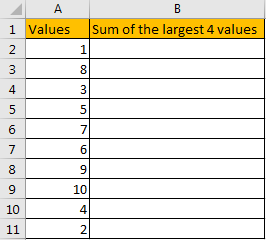In the ‘Values’ column, there are 10 values from ‘1’ to ‘10’ in improper order. Now we want to sum the largest 4 values from this list, how can we get the correct result?

Actually, we can sort these numbers from the largest to the smallest by ‘Sort Z-A’ in excel.

Click ‘Data’ tab, under ‘Sort & Filter’ section click ‘Sort Z to A’ icon.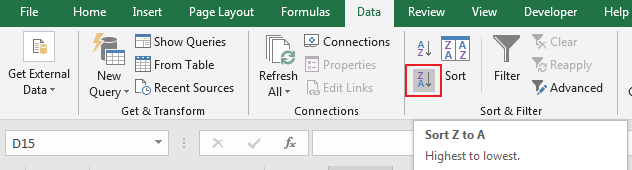Then you can find numbers are ordered properly.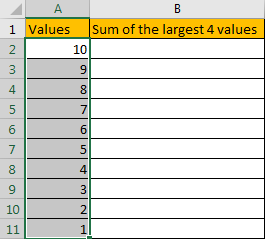Then you can enter SUM function in B2 and select the first four numbers ->cell reference A2:A5 in the list.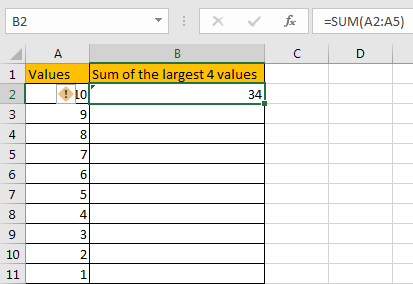Then you can get the sum of the largest four values. It is 34.

In this way, we can get result correctly. But we can also get it conveniently and correctly by just enter a formula. As we want to sum the largest four numbers from range A2:A11, we can find the largest N number or numbers by LARGE function actually, and then use SUMPRODUCT function to sum array directly.

## FORMULA – APPLY SUMPRODUCT FUNCTION

Step 1: In B2, enter the formula

` =SUMPRODUCT(LARGE(A2:A11,{1,2,3,4})).`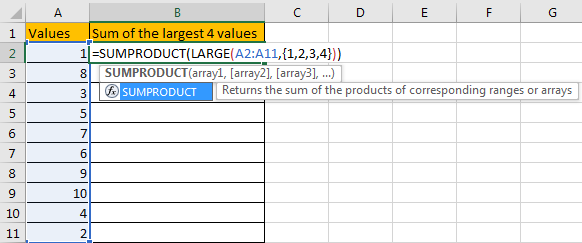Step 2: Press Enter after typing the formula.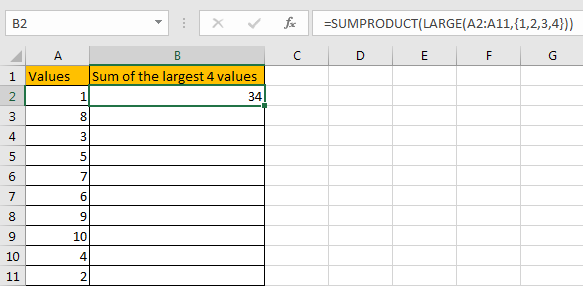We can see that this time we also get correct result 34. The formula works correctly.

## FUNCTION INTRODUCTION

SUMPRODUCT function can be seen as SUM+PRODUCT.

For SUMPRODUCT function, the syntax is:

`=SUMPRODUCT(array1,array2,array3, ...)`

For example, enter =SUMPRODUCT({1,2},{2,3}) in any cell, the we can get value 8, it equals to 1*2+2*3=8. You can also enter =SUMPRODUCT({1,2}*{2,3}), you can get the same result. The argument array can be a real array {1,2,3} for example or a range reference like A2:A7.

If there is only array in the formula, SUMPRODUCT will sum the numbers in the array.

SUMPRODUCT function allows entering texts, logical operators and expressions like (product=cap), texts should be enclosed into ().

LARGE function can return the Nth largest value in a range.

For LARGE function, the syntax is:

`=LARGE(array,k)`

If k is a number, it returns the Kth largest value in the range; if k is an array like {1,2,3}, it returns the largest three values in the range. If k is an array, but the numbers are in inconsecutive sequence for example k={1,2,4}, then LARGE function will return the 1st, 2nd, 4th largest values in the range.

## ARGUMENTS EXPLAINATION

In this formula =SUMPRODUCT(LARGE(A2:A11,{1,2,3,4})), we applied two functions.

For LARGE function, range is A2:A11, k is an array {1,2,3,4}.

Select range A2:A11 in formula bar, press F9 to convert cell reference to real values, numbers in ‘Values’ column are expanded in an array.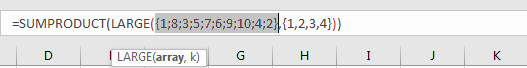For SUMPRODUCT function, there is only one array, it is the result of formula LARGE({1;8;3;5;7;6;9;10;4;2},{1,2,3,4}).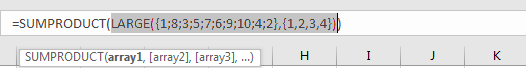## HOW THIS FORMULA WORKS

After explaining argument in the formula, the formula is converted to =SUMPRODUCT(LARGE({1;8;3;5;7;6;9;10;4;2},{1,2,3,4})) in the formula bar. Now we will show you how the formula works with the two functions.

For LARGE({1;8;3;5;7;6;9;10;4;2},{1,2,3,4}), refer to k value {1,2,3,4}, we find out the largest 4 values in the array {1;8;3;5;7;6;9;10;4;2}.

Select LARGE({1;8;3;5;7;6;9;10;4;2},{1,2,3,4}) in the formula bar, press F9. We can get the result {10,9,8,7}. Numbers are ordered from the largest to the smallest.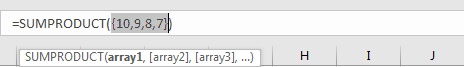Obviously, the final result is 10+9+8+7=34. Select =SUMPRODUCT({10,9,8,7}) in the formula bar, press F9, 34 is displayed in formula bar.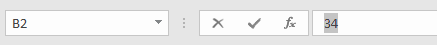1. In this instance, there are only 10 numbers in the range, and we only want to sum the largest 4 numbers. If there are a large amount of numbers, and sum the largest 100 or more numbers in the range, do we need to enter {1,2,3,4,…,100} in LARGE function? Obviously, it is very complex and troublesome. So, we can solve this problem by the help of ROW and INDIRECT

For example, if we want to sum the largest 9 numbers in the same range, we can enter the follow formula in Cell B2:

`=SUMPRODUCT(LARGE(A2:A11,ROW(INDIRECT("1:9"))))`

Then we can get correct value 54.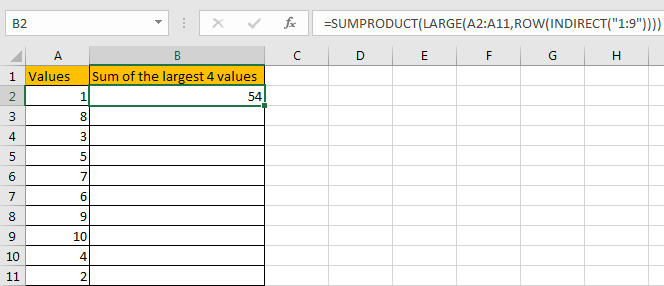In this case, INDIRECT function can return a valid reference based on entered texts. With the help of ROW, ROW(INDIRECT(“1:9”)) can convert “1:9” to {1,2,3,4,5,6,7,8,9}.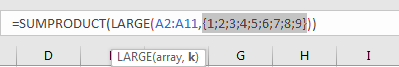2. If N number is saved in another cell, we can concentrate N inside INDIRECT function. Enter the follow formula in Cell d2 this time:

`=SUMPRODUCT(LARGE(A2:A11,ROW(INDIRECT("1:"&D1))))`

See example below: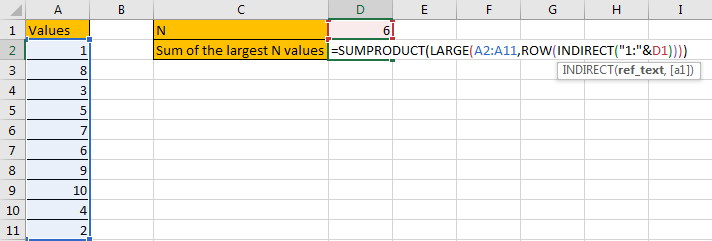In this case, when changing the number in D1, result in D2 will be automatically updated. We don’t need to adjust the formula or cell reference. See example below.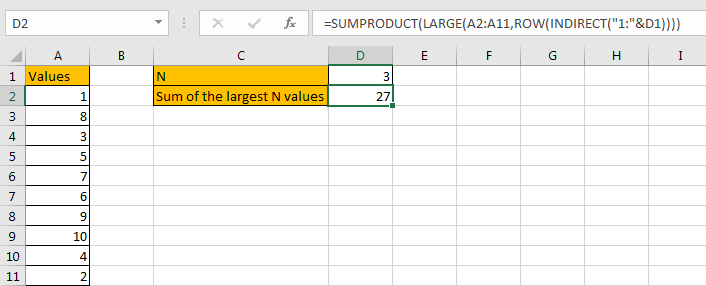3. In this case we can also use SUM function to replace SUMPRODUCT function. But SUMPRODUCT function can handle arrays directly without entering Ctrl + Shift + Enter, so we often apply SUMPRODUCT function in similar situations.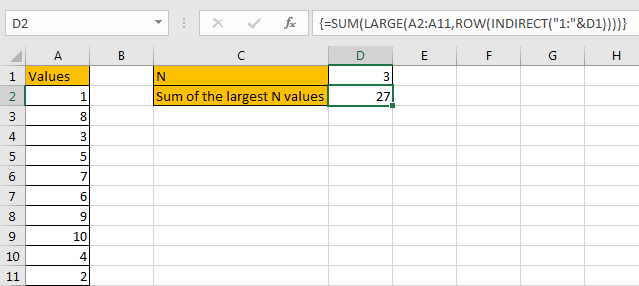SUMMARY

1. SUMPRODUCT function can handle multiple arrays, and it sum up the products.

2. SUMPRODUCT function allows user defined range, wildcards, logical operators, expressions.

3. LARGE function can return the Nth or first Nth largest value in a range.

4. ROW(INDIRECT(“1:N”)) can return a set of numeric numbers in an array.

5. If N is in another cell, use “&” to concentrate them inside INDIRECT, for example ROW(INDIRECT(“1:”&B2)).

### Related Functions

• Excel SUMPRODUCT function
The Excel SUMPRODUCT function multiplies corresponding components in the given one or more arrays or ranges, and returns the sum of those products. The syntax of the SUMPRODUCT function is as below:= SUMPRODUCT (array1,[array2],…)…
• Excel ROW function
The Excel ROW function returns the row number of a cell reference.The ROW function is a build-in function in Microsoft Excel and it is categorized as a Lookup and Reference Function.The syntax of the ROW function is as below:= ROW ([reference])….
• Excel LARGE function
The Excel LARGE function returns the largest numeric value from the numbers that you provided. Or returns the largest value in the array. The syntax of the LARGE function is as below:= LARGE (array,nth)…
• Excel INDIRECT function
The Excel INDIRECT function returns the cell reference based on a text string, such as: type the text string “A2” in B1 cell, it just a text string, so you can use INDIRECT function to convert text string as cell reference….
• Excel SUM function
The Excel SUM function will adds all numbers in a range of cells and returns the sum of these values. You can add individual values, cell references or ranges in excel.The syntax of the SUM function is as below:= SUM(number1,[number2],…)

## How to Auto Fill Weekdays or Weekends in Excel

Sometimes we may want to enter a sequence of days in excel for some purpose, and we can press Ctrl+; to insert current date into cell, and then drag the cell down to attach following days into other cells, then we can get a list of days.

But for some special requirement, we just want to fill weekdays or weekends into a list, for example if weekdays exist in the list, weekends are excluded. So, we need to find a way to only insert weekdays or weekends simply. In this article, we will show you the ways to fill days into list per your requirement properly, we provide two ways to fill weekdays or weekends, by Auto Fill function or the formula.

## Method 1: Auto Fill Weekdays in Excel

Step 1: Launch excel open a new worksheet, enter the start date into any cell, if you want to fill only weekdays into the list, please enter a weekday, then drag it down to the next cells till we get the end date in date list. For example, we want to fill weekdays from 9/1/2020 to 9/15/2020, then enter 9/1/2020 in any cell, then drag it down to auto fill days till we get 9/15/2020.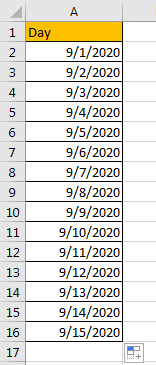Step 2: You may notice that when we dragging the cell down to apply formula to other cells, Auto Fill Options icon is displayed and attached to the last cell. Click on Auto Fill Options icon to load all options. Verify that ‘Fill Weekdays’ option is included.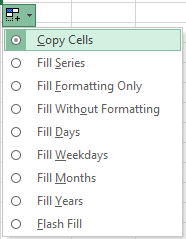Step 3: Check on ‘Fill Weekdays’ option, verify that days are updated from previous 9/1/2020 to 9/21/2020 automatically, that’s because weekends are removed from the list and weekdays are filled instead.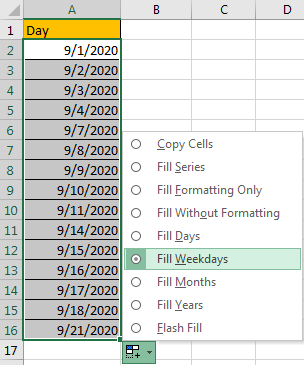Step 4: If you want to keep the end date as 9/15/2020 in your list, just remove the extra days from the list. Now date list with only weekdays is created properly.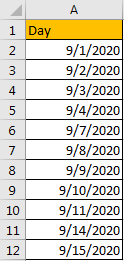## Method 2: Auto Fill Weekends by Formula in Excel

You can see that there is no fill weekends option in Auto Fill Options list. So, we need to find another way to fill weekends only. Actually, you can through below formula to fill weekends properly.

Step 1: Launch excel open a new worksheet, enter the formula =TEXT(“09/05/2020″+INT(ROW(A1)/2)+(CEILING(ROW(A1)/2,1)-1)*6,”mm/dd/yyyy”) into any cell. As you want to fill only weekends into the list, please enter a weekend date into the formula, in this sample we enter 09/05/2020 as start date, you can also change date format ‘mm/dd/yyyy’ to another format.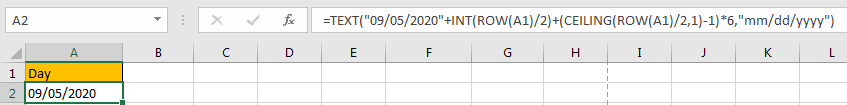Step 2: Then drag it down to the next cells to apply the formula. Till we get the end date in date list. For example, we want to fill all weekends in September, drag it down till weekend date from October occurs.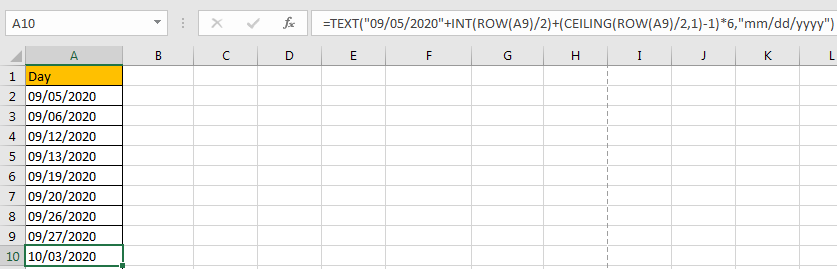Step 3: Just remove the date from October, then weekends from September are listed.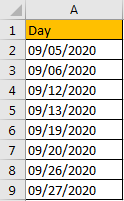### Related Functions

• Excel ROW function
The Excel ROW function returns the row number of a cell reference.The ROW function is a build-in function in Microsoft Excel and it is categorized as a Lookup and Reference Function.The syntax of the ROW function is as below:= ROW ([reference])….
• Excel INT function
The Excel INT function returns the integer portion of a given number. And it will rounds a given number down to the nearest integer.The syntax of the INT function is as below:= INT (number)…
• Excel Text function
The Excel TEXT function converts a numeric value into text string with a specified format. The TEXT function is a build-in function in Microsoft Excel and it is categorized as a Text Function. The syntax of the TEXT function is as below: = TEXT (value, Format code)…
• Excel Ceiling function
The Excel CEILING function returns a given number rounded up to the nearest multiple of a given number of significance.The syntax of the CEILING function is as below:= CEILING (number, significance)